# 前言

1. 每个节点要么是黑色，要么是红色。
2. 根节点是黑色。
3. 每个叶子节点（NIL）是黑色。（这一点在HashMap里并没有去实现NIL节点的，所以HashMap里的叶子节点就是我们正常理解的叶子节点）
4. 每个红色结点的两个子结点一定都是黑色。（这一点可以得到一个很有用的结论：已平衡的情况下，一个红色节点，它的parent、left和right都为黑色）
5. 任意一结点到每个叶子结点的路径都包含数量相同的黑结点。（这一点在每一次的插入和删除时保证）

# root

        final TreeNode<K,V> root() {
for (TreeNode<K,V> r = this, p;;) {
if ((p = r.parent) == null)
return r;
r = p; //r往上移动，继续下一次循环
}
}


# moveRootToFront

        static <K,V> void moveRootToFront(Node<K,V>[] tab, TreeNode<K,V> root) {
int n;
if (root != null && tab != null && (n = tab.length) > 0) {
int index = (n - 1) & root.hash;
TreeNode<K,V> first = (TreeNode<K,V>)tab[index];//得到当前table下标指向的节点
if (root != first) {//如果该table下标的第一个节点都已经是入参root了，就不用进分支了
Node<K,V> rn;//r的next
tab[index] = root;//将table下标指向root
TreeNode<K,V> rp = root.prev; //因为root不是first，所以它的prev肯定不为空
if ((rn = root.next) != null)  //那么root也不是双向链表的尾节点
((TreeNode<K,V>)rn).prev = rp;
if (rp != null)
rp.next = rn;
if (first != null)
first.prev = root;
root.next = first;
root.prev = null;
}
assert checkInvariants(root);
}
}

• TreeNode有parent、left、right成员，所以它可以作为红黑树节点。TreeNode有prev、next成员，所以它也可以作为双向链表节点。正常状态下，作为红黑树存在的哈希桶，它的第一个节点肯定也是该红黑树的root节点。但是在经过结构化修改后（增加或删除节点），红黑树会自我调整以至于哈希桶的第一个节点不再是root了。此时，需要调用moveRootToFront函数。
• 想象作为红黑树存在的哈希桶，我们观察它时，既可以用红黑树的视图来看，也可以用双向链表的视图。而此函数的作用就是，改变该哈希桶的双向链表，使得root变成双向链表的头结点。注意，双向链表的头结点的prev为null，尾结点的next为null。这个函数干的事其实大概就是下图（只是为了理解，实际执行顺序请看代码，画图我偷懒了，双向箭头其实应该画成两个箭头）：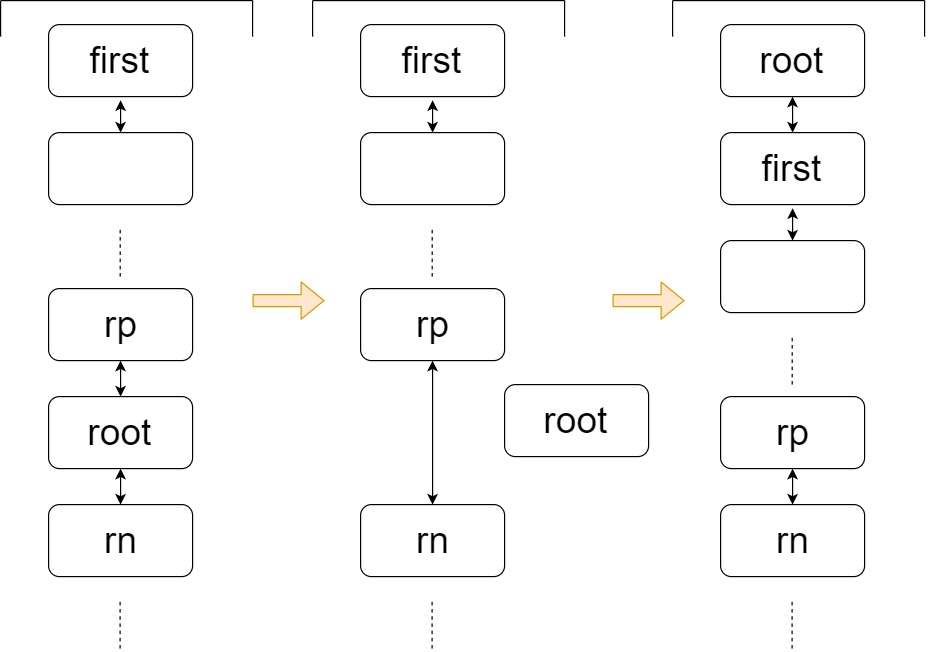# find

Finds the node starting at root p with the given hash and key. The kc argument caches comparableClassFor(key) upon first use comparing keys.

        final TreeNode<K,V> find(int h, Object k, Class<?> kc) {
TreeNode<K,V> p = this;
do {
int ph, dir; K pk;
TreeNode<K,V> pl = p.left, pr = p.right, q;
if ((ph = p.hash) > h) //如果传入节点的hash值比当前节点p小，那么进入左子树
p = pl;
else if (ph < h) //如果传入节点的hash值比当前节点p大，那么进入右子树
p = pr;
else if ((pk = p.key) == k || (k != null && k.equals(pk)))//如果是同一个引用或equals判定相等
return p;//认为找到了相同节点，终点。
//如果执行到这里，说明传入节点的hash值与当前相等，且没有通过equals判定
else if (pl == null)
p = pr;
else if (pr == null)
p = pl;
//下面是短路或。如果kc不为null，前式子成立，不会执行后面；如果kc为null，前式子不成立，执行后面。
//这说明如果传入的kc不为null，则肯定是Comparable接口的自限定的类的Class
else if ( (kc != null || (kc = comparableClassFor(k)) != null) &&
(dir = compareComparables(kc, k, pk)) != 0 )//如果Comparable比较后，传入节点跟当前节点不一样大(!= 0)
p = (dir < 0) ? pl : pr;//如果传入节点的hash值比当前节点p小，那么进入左子树;否则，进入右子树
//执行到这里说明，要么类型参数K的类定义不是Comparable的自限定；要么Comparable比较后，判定相等
//所以要想找到传入节点，要么在左子树找，要么在右子树找
else if ((q = pr.find(h, k, kc)) != null)//左子树若找不到，就返回null。此时，进入最后的else分支
return q;
else
p = pl;
//执行到这里，说明p已经被更新为它的左孩子或右孩子了
} while (p != null);//如果更新后发现为null，说明按照二叉树查找后，没有找到
return null;//终点
}

• 此函数用来找到与传入节点k相同的那个既存节点，整个查找过程是二叉树的查找过程。此函数是递归函数。
• 如果传入节点k的hash值与当前节点p不一样，那么相同的既存节点肯定在左子树或右子树里。
• 如果传入节点k与当前节点p判断相等，那么返回当前节点，这是该递归函数的终点之一。
• 如果传入节点k的hash值与当前节点p一样，且equals判定不相同，那么此时，如果左右子树有一个为null，那么只能走另外一条路。
• 如果传入节点k的hash值与当前节点p一样，且equals判定不相同，且左右子树都不为null，那么此时，如果通过Comparable比较后比出了大小（返回结果不是0），那么按照大小结果进入左或右。
• 如果传入节点k的hash值与当前节点p一样，且equals判定不相同，且左右子树都不为null，且Comparable比不出大小（还可能是类定义不是Comparable的自限定，所以比不了），那么此时，再也没有捷径了，只能左右子树都去寻找一下（左子树是递归去寻找，右子树则是在左子树递归为null后，在当前函数继续循环来寻找）。
• 如果循环体结束后，while判断条件发现p为null，则说明已经找到了叶子节点（左右孩子都为null）（具体情况是，判断了else if (pl == null)，发现成立，然后执行了p = pr;），说明没有找到以参数k作为key的mapping。

# getTreeNode

        final TreeNode<K,V> getTreeNode(int h, Object k) {
//如果自身不是root，则先找到root
return ((parent != null) ? root() : this).find(h, k, null);
}


# tieBreakOrder

Tie-breaking utility for ordering insertions when equal hashCodes and non-comparable. We don’t require a total order, just a consistent insertion rule to maintain equivalence across rebalancings. Tie-breaking further than necessary simplifies testing a bit.

        static int tieBreakOrder(Object a, Object b) {
// 不能返回0，因为必须要tieBreak，分出个大小
int d;
// 如果，a为null，或者b为null，或者a对象的类名和b对象的类名一样（即是同一个类的对象）：就会进入if分支
// 如果a对象的类名和b对象的类名通过字符串比较得出大小不是0，那么不会进入if分支，且d会被赋值
// 进入if分支，说明必须使用identityHashCode，即使hashCode被重写，这个方法也会返回原生的hashCode方法的值
if (a == null || b == null ||
(d = a.getClass().getName().
compareTo(b.getClass().getName())) == 0)
// 当a和b指向同一个对象，identityHashCode会相等。即使这种情况，也认为a小于b，因为必须分出个大小
d = (System.identityHashCode(a) <= System.identityHashCode(b) ?
-1 : 1);
return d; //-1代表a小，1代表a大
}


# treeify

        final void treeify(Node<K,V>[] tab) {
TreeNode<K,V> root = null;
for (TreeNode<K,V> x = this, next; x != null; x = next) { //循环最后会让x指向next，然后在下一次循环里处理新x
next = (TreeNode<K,V>)x.next; //先把x的后继保存到next，这里转型是因为Node父类的next引用为Node，所以要转回来
x.left = x.right = null;
//x就是当前循环处理的节点。如果此时根节点还没分配，那么将x作为root
if (root == null) {
x.parent = null;
x.red = false; //root为黑色
root = x;
}
// 进入else说明root已经有了
// 下面的x肯定是第二个元素或更后面的元素
else {
K k = x.key;
int h = x.hash;
Class<?> kc = null;  //key的Class对象
for (TreeNode<K,V> p = root;;) { //初始让p指向root
int dir, ph;
K pk = p.key;
if ((ph = p.hash) > h)
dir = -1; //代表当前处理节点x，比p小
else if (ph < h)
dir = 1; //代表当前处理节点x，比p大
// 如果hash值一样，则只能通过别的方式分出个大小
else if ((kc == null && //如果kc之前没有被赋值过，注意它与下一排在一个括号里
(kc = comparableClassFor(k)) == null) || //如果的k的类定义是Comparable的泛型自限定，那么赋值给kc
(dir = compareComparables(kc, k, pk)) == 0) //执行到这里说明k的类定义是Comparable的泛型自限定，且kc已经赋值为其Class对象
//如果pk与k类型一样，那么返回k.compareTo(x)；否则返回0，所以返回0既可能代表k和pk一样大，也可能是二者没法比
dir = tieBreakOrder(k, pk); //返回-1或者1

TreeNode<K,V> xp = p;//让xp指向p，因为p引用马上要被替换掉了
// 根据dir的正负让p指向p的左孩子或右孩子，如果孩子不为null，继续下一次循环；否则进入分支
// 只有进入if分支才能退出循环（里面有break），整个循环过程就是二叉查找的过程，直到找到叶子节点
// 循环过程中，p会根据与x的比较结果一直向下移动
if ((p = (dir <= 0) ? p.left : p.right) == null) {
//通过二叉查找找到了x的最终位置，然后将xp（x的parent）与x连接起来
x.parent = xp;
if (dir <= 0)
xp.left = x;
else
xp.right = x;
//注意x并没有给red变量赋过值
root = balanceInsertion(root, x);
break;
}
}
}
}
moveRootToFront(tab, root);
}

• 会在TreeNode对象形成的双向链表的头节点上，调用这个成员方法。既然有了头节点，那么就能获取到该双向链表的所有节点，该函数遍历节点，通过改变TreeNode对象的红黑树相关成员，使得各个节点形成红黑树结构。
• 整个函数由双层循环构成。外层循环负责遍历双向链表（x为当前处理节点，循环的运作靠next = (TreeNode<K,V>)x.nextx = next），如果root节点还没有分配，那么分配root节点，否则把活交给内层循环。
• 进入内层循环说明红黑树中已经至少有一个节点了，此时要放置个新节点进去。首先要二叉查找树的过程，找到新节点的放置位置，整个过程为：
1. dir会得到一个非0值（-1代表当前处理节点x更小，所以要进入左子树，+1反之，则进入右子树），(dir <= 0) ? p.left : p.right这里根据非0值把左孩子或右孩子赋值给p，继续下次循环；
2. 重复这个下降过程；
3. 当找到放置的位置时，if ((p = (dir <= 0) ? p.left : p.right) == null)分支会发现p会被赋值为null，因为p经过若干次循环后已经到达了一个叶子节点。此时每次用来保存原p引用的xp终于派上了用场（因为p会被置为null，不提前保存，这个引用就丢了）；
4. 但现在只是按照二叉查找的过程找到了一个位置而已，这个位置是暂时的，因为新节点放在这里不一定符合红黑树结构，所以需要调用balanceInsertion，调用完毕，才算完成。
• 当双层循环执行完毕后，需要调用moveRootToFront来使得root节点作为双向链表的头结点。

# untreeify

        final Node<K,V> untreeify(HashMap<K,V> map) {
//this对象已经是双链表的第一个节点了
Node<K,V> hd = null, tl = null;
for (Node<K,V> q = this; q != null; q = q.next) {
Node<K,V> p = map.replacementNode(q, null);//new出普通node来
if (tl == null)
hd = p;
else
tl.next = p;
tl = p;
}
return hd;
}

• 调用此函数时，this对象已经是双链表的第一个节点了。

# putTreeVal

        final TreeNode<K,V> putTreeVal(HashMap<K,V> map, Node<K,V>[] tab,
int h, K k, V v) {
Class<?> kc = null;
boolean searched = false;//一定需要这个变量？
TreeNode<K,V> root = (parent != null) ? root() : this;//parent是被调动putTreeVal成员函数的那个节点的父节点（成员）
for (TreeNode<K,V> p = root;;) {
int dir, ph; K pk; //dir代表传入节点与当前循环节点的比较大小
if ((ph = p.hash) > h) //如果传入节点的hash比当前处理节点小
dir = -1;
else if (ph < h) //如果传入节点的hash比当前处理节点大
dir = 1;
//如果传入节点的hash跟当前处理节点一样大，传入key与当前key判定相等，那么返回相同节点
else if ((pk = p.key) == k || (k != null && k.equals(pk)))
return p;
//如果传入节点的hash跟当前处理节点一样大，传入key与当前key判定不相等，但又必须比出个大小来
//所以要么使用comparable接口，要么使用tieBreakOrder，最终dir会被赋值一个非0值
//分支进入条件1：如果类型参数K的类定义不是comparable接口的自限定
//分支进入条件2：虽然类型参数K的类定义是comparable接口的自限定，但传入节点和当前节点比较后为0
//总之这两种情况都没有比出大小
else if ((kc == null && (kc = comparableClassFor(k)) == null) ||
(dir = compareComparables(kc, k, pk)) == 0) {
if (!searched) {//这个分支只会进入一次，因为searched马上被置为true了
TreeNode<K,V> q, ch;
searched = true;
if (((ch = p.left) != null &&
(q = ch.find(h, k, kc)) != null) || //先去左子树找
((ch = p.right) != null &&
(q = ch.find(h, k, kc)) != null)) //先去右子树找
//如果能找到就返回相同节点，如果不能找到那说明整个红黑树里面没有相同节点，因为都找遍了
//所以if (!searched)只能进入一次，当然if (!searched)分支也可能一次都不进入，
//当传入节点与红黑树内所有节点都能比较出大小时
return q;
}
//执行到这里说明if (!searched)分支已经执行过了，且没有整棵树都没有找到相同节点
//但还是必须从左右子树挑一条路走，那么使用tieBreakOrder
dir = tieBreakOrder(k, pk); //为保持一致，它与treeify里tieBreakOrder的实参顺序一样
}
//至此，dir已得到一个非0值
TreeNode<K,V> xp = p; //xp暂存p引用，以备不时之需
//p根据dir更新为它自己的左孩子或右孩子，更新后不为null则继续循环，为null则代表找到了最终插入位置
if ((p = (dir <= 0) ? p.left : p.right) == null) {
Node<K,V> xpn = xp.next; //注意还需要维护双链表结构
TreeNode<K,V> x = map.newTreeNode(h, k, v, xpn); //new一个TreeNode实例
//赋值父节点的孩子指针
if (dir <= 0)
xp.left = x;
else
xp.right = x;
xp.next = x; //赋值链表结构的后继指针
x.parent = x.prev = xp;
if (xpn != null)
((TreeNode<K,V>)xpn).prev = x;
//执行到这里，只是按照二叉查找的过程暂时放置了传入节点，为保持红黑树性质，
//需调用balanceInsertion，调用后返回新root（如果root发生了改变），
//再调用moveRootToFront，使得数组下标能指向红黑树的root节点
moveRootToFront(tab, balanceInsertion(root, x));
return null;//既然已经新增了节点，所以返回null
}
}
}

• 阅读完源码你会发现，整个函数的流程与我们的猜想一模一样。
• 整个流程在for循环里，将p节点按照二叉查找的过程往下移动（每次循环会更新p），移动的方向根据变量dir的正负来往下走，如果整棵树里都没有与传入节点相同的既存节点，那么最终肯定会找到新节点的插入位置（if ((p = (dir <= 0) ? p.left : p.right) == null)分支）：进入分支后，先new出一个TreeNode实例，再与周围节点重塑好双链表结构和红黑树结构，最后再做红黑树的自我调整以保持保持红黑树性质。
• 当传入节点与当前比较节点p使用comparable接口都比较不出大小来时（else if ((kc == null && (kc = comparableClassFor(k)) == null) || (dir = compareComparables(kc, k, pk)) == 0)分支），就会进入if (!searched) {分支，这个分支只会进入一次，但进入后会把p的左右子树都搜索一遍，如果整棵树里面都没有相同节点，那么说明这个传入节点肯定会被插入，但还是需要先找到个插入位置，所以调用tieBreakOrder来获得默认顺序。
• 这里解释一下为什么if (!searched) {分支里，p的左右子树都搜索一遍：
• 虽然tieBreakOrder会给无法比较出大小的两个节点以默认顺序，但是由于还有左旋右旋操作，所以两个无法比较出大小的节点在红黑树中的顺序可能会被破坏。
• 默认顺序是新插入节点即使比较不出大小，也会把新插入节点放在左孩子（即认为新插入的更小），但由于之后还有红黑树的自我调整，可能会用到旋转操作，那么两个节点的孩子关系可能会变成右孩子的关系。
• 而且这种比较不出大小的情况可能会多次发生，那么干脆在遇到这种情况时，把当前p的左右子树都去找一遍得了（因为find函数会递归查找树上的所有节点），但这样肯定比较耗时，所以要留到第一次遇到比较不出大小的情况才执行这种操作。
• 当然，if (!searched) {分支也可能一次都不会进入，如果传入节点在二叉查找的过程中，与路径上的所有节点都能比较出大小（不管是用hash值，还是用comparable接口）。因为这种情况，二叉查找路径一直都是明确的（该往左子树走，还是该往右子树走）。

# removeTreeNode

Removes the given node, that must be present before this call. This is messier than typical red-black deletion code because we cannot swap the contents of an interior node with a leaf successor that is pinned by “next” pointers that are accessible independently during traversal. So instead we swap the tree linkages. If the current tree appears to have too few nodes, the bin is converted back to a plain bin. (The test triggers somewhere between 2 and 6 nodes, depending on tree structure).

        final void removeTreeNode(HashMap<K,V> map, Node<K,V>[] tab,
boolean movable) {
//进入此函数后，this就是那个要被删除的节点了
int n;
if (tab == null || (n = tab.length) == 0)
return;
int index = (n - 1) & hash;//获得this节点的所在数组下标
TreeNode<K,V> first = (TreeNode<K,V>)tab[index], root = first, rl;//first代表双向链表头节点；root代表红黑树根节点
TreeNode<K,V> succ = (TreeNode<K,V>)next, pred = prev;//this节点的前驱后继
if (pred == null)//如果被删节点是root
tab[index] = first = succ;//用root的后继来更新该数组下标，且更新first。这样从双向链表上看，该节点已经被删除
else//如果被删节点不是root
pred.next = succ;//更新前驱节点的后继
if (succ != null)//如果后继节点不为null
succ.prev = pred;//更新后继节点的前驱
//执行到这里，说明排除了上面说的特殊情况。此时，从双向链表的视图来看，该节点已经被删除，因为已经修改好了前驱后驱了

//有两种情况直接返回。1.若被删节点是root(进入if (pred == null)分支)，且root节点的后继为null，这说明红黑树里只有一个节点（前驱后继都为null）
//这种情况下，tab[index]会被置为null，所以是正确的操作
//2.若被删节点不是root，但root引用最初被table下标赋值时是null，这种情况看起来有点奇怪，因为好像不可能发生
//毕竟这种情况是该table下标指向了null，代表该哈希桶里没有元素
if (first == null)
return;
if (root.parent != null)//排除root引用不是红黑树的根节点的特殊情况
root = root.root();
//下面分支排除了一些直接返回的情况，总之此时认为树内节点太少，不需要保持红黑树结构了，直接untreeify后返回即可：
//1.root为null    2.root不为null，但root.right为null   3.root和root.right都不为null，但root.left为null
//4.root和root.right和root.left都不为null，但rl.left为null
if (root == null || root.right == null ||
(rl = root.left) == null || rl.left == null) {
tab[index] = first.untreeify(map);  // too small
return;
}
TreeNode<K,V> p = this, pl = left, pr = right, replacement;
//p为删除节点
if (pl != null && pr != null) {//如果p的两个孩子都不为null，这种情况最复杂
TreeNode<K,V> s = pr, sl;
while ((sl = s.left) != null) // find successor找到右子树的最左节点
s = sl;
boolean c = s.red; s.red = p.red; p.red = c; // swap colors交换s和p的颜色
TreeNode<K,V> sr = s.right;
TreeNode<K,V> pp = p.parent;
if (s == pr) { // p was s's direct parent
p.parent = s;
s.right = p;
}
else {
TreeNode<K,V> sp = s.parent;
if ((p.parent = sp) != null) {
if (s == sp.left)//s既然是最左节点，所以肯定是左孩子啦
sp.left = p;
else
sp.right = p;
}
if ((s.right = pr) != null)
pr.parent = s;
}
//以下几句肯定执行
p.left = null;
if ((p.right = sr) != null)//如果sr不为null，拼接p与sr
sr.parent = p;
if ((s.left = pl) != null)//如果pl不为null，拼接s与pl
pl.parent = s;
//这里区别pp的情况
if ((s.parent = pp) == null)
root = s;
else if (p == pp.left)
pp.left = s;
else
pp.right = s;
//这里区分sr的情况
if (sr != null)
replacement = sr;
else
replacement = p;
}
else if (pl != null)//只有pl不为null
replacement = pl;
else if (pr != null)//只有pr不为null
replacement = pr;
else
replacement = p;
//这里已经得到了replacement，判断replacement的情况
if (replacement != p) {
TreeNode<K,V> pp = replacement.parent = p.parent;
if (pp == null)
root = replacement;
else if (p == pp.left)
pp.left = replacement;
else
pp.right = replacement;
p.left = p.right = p.parent = null;
}

TreeNode<K,V> r = p.red ? root : balanceDeletion(root, replacement);

if (replacement == p) {  // detach
TreeNode<K,V> pp = p.parent;
p.parent = null;
if (pp != null) {
if (p == pp.left)
pp.left = null;
else if (p == pp.right)
pp.right = null;
}
}
if (movable)
moveRootToFront(tab, r);
}

• 进入此函数时，this指向的就已经是那个需要被删除的节点。
• 执行完if (succ != null) succ.prev = pred;这句，通过连接this节点的prev节点和next节点，使得在双链表的视图下，this对象已经被删除掉了。但红黑树的视图下，this还没有被删除掉。
• 如果当前红黑树内的节点太少，则进入if (root == null || root.right == null || (rl = root.left) == null || rl.left == null)分支，然后调用untreeify方法，这说明untreeify方法里只会利用双链表相关的成员（prev和next）就够了。注意这里必须是first引用来调用untreeify方法，因为经过之前的处理后，first肯定是双链表视图的下的第一个节点，且双链表视图下删除节点已经被删除掉了。

• 提前说明一下，当说到“x节点子树的黑节点数n”是指：从x节点到它的子树的任意一个叶子节点的路径上的黑色节点个数都等于n。
• 第一种情况：pl和pr都为null，此时进入else if (pr != null)的else分支，并执行replacement = p。由于p没有孩子，所以是直接删除掉p节点就行。而replacement是个很重要的引用，将replacement传入balanceDeletion时，则代表replacement节点子树的黑节点数，比replacement的兄弟节点子树的黑节点数少1，所以传入时replacement与其兄弟节点之间已经不平衡了。
• 然后不会进入if (replacement != p)分支，直接执行TreeNode<K,V> r = p.red ? root : balanceDeletion(root, replacement)。很好理解，p是要删除的那个节点，如果p是红色的，删除p后各路径的黑色节点数还是一样，不用做平衡操作。如果p是黑色，删除后必将不平衡，所以需要平衡操作。
• 而在balanceDeletion函数调用后，还有一个if (replacement == p)分支，第一种情况刚好会走此分支，此分支的逻辑就是令p和p的父节点之间断绝关系，实际就是执行红黑树视图下的删除操作（修改left、right、parent引用来达到）。
• 如果balanceDeletion函数调用后，replacement == p成立的话，说明之前的逻辑还没有执行过红黑树视图下的删除操作，或者说之前还不可以做删除操作，因为replacement要作为一个与其兄弟节点间不平衡的节点传入balanceDeletion，如果之前就删除，就没法传入balanceDeletion函数了。虽然之前还没删除，但之后也会删除掉的，所以可以认为replacement比其兄弟节点的黑节点数少1。
• 第二种情况或第三种情况：pl和pr有一个null，由于这两种情况完全对称，所以只分析一个。假设此时进入else if (pl != null)分支，并执行replacement = pl。此时会进入if (replacement != p)分支，其流程如下图，可见，最终效果是将pp和pl连接起来，并使得p节点脱离了红黑树。p节点脱离了红黑树，其实就是执行红黑树视图下的删除操作，既然已经将p节点进行了删除，所以之后不需要也不会进入if (replacement == p)分支。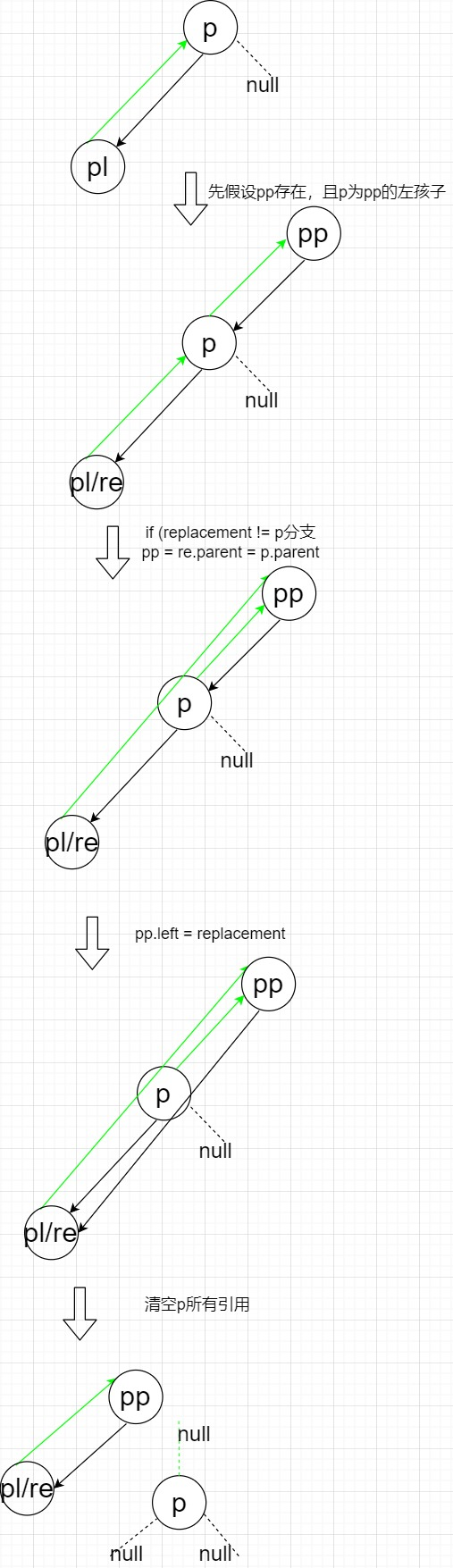• 上图假设pp存在，且p为pp左孩子。其他情况分析是类似的：如果p为pp右孩子，那么最终re与pp会保持右孩子关系。如果pp不存在，那么最终结果里，re的父节点为null。
• balanceDeletion函数调用前，会进入到if (replacement != p)的分支（以执行红黑树视图的删除操作）。balanceDeletion函数调用后，不会进入到if (replacement == p)的分支（因为不会进入代表之前已经执行了删除操作）。
• 最后分析最难的情况，pl和pr都不为null。要理解此情况需要理解这个“重要观点”：不考虑颜色的情况下，删除掉删除节点等同于“将删除节点的后继节点作为替换节点替换到删除位置上去”，这里的后继指的是刚好大于删除节点的那个节点。由于替换节点会换位置，且删除位置还将拥有节点，所以其实相当于删除掉了替换节点。

• p为删除节点
• 执行完while ((sl = s.left) != null)循环，s会指向p的后继（刚好大于p的那个节点），而且这能保证s节点肯定没有左孩子，因为它已经是p的右子树的最左节点了。所以s为替换节点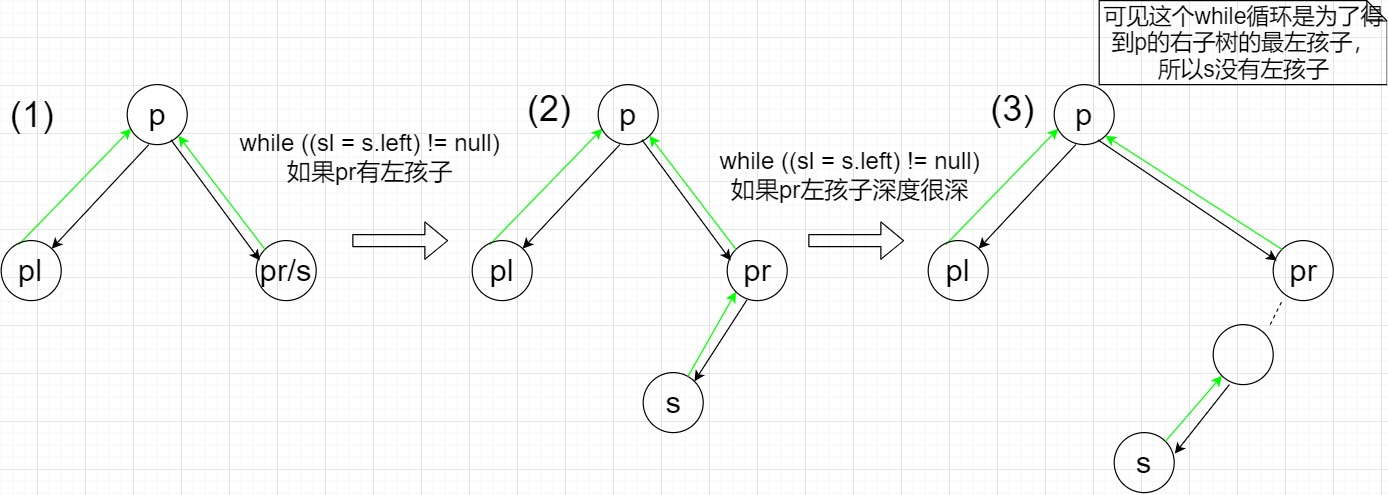• 为了方便理解，我们假设当前的情况为过程$(1)$，即p的后继就是pr。下面大图包括了所有过程。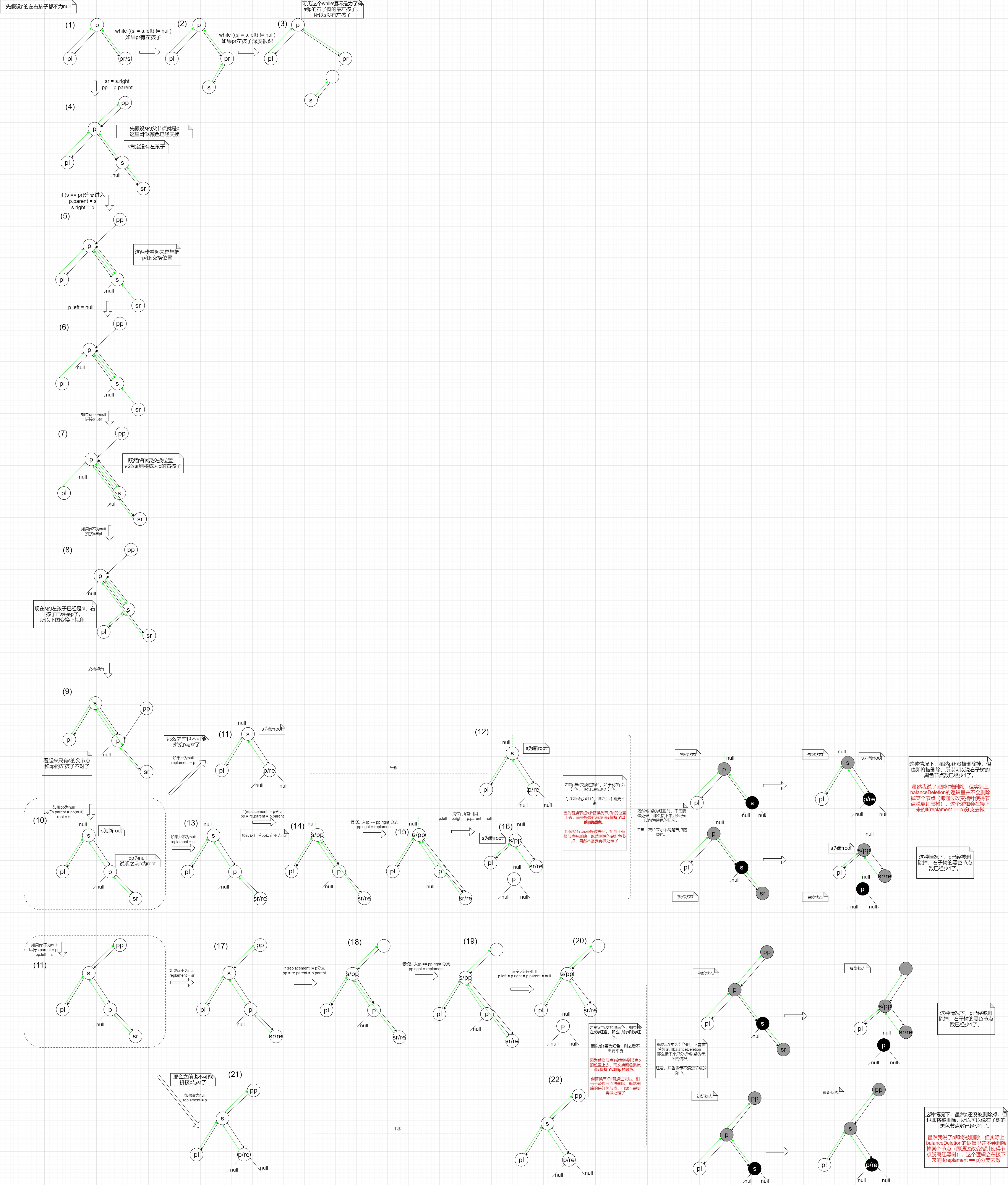• boolean c = s.red; s.red = p.red; p.red = c;这句会交换p和s的颜色，因为s最终会跑到p的位置上来，执行了这句能使得s能保持之前p的颜色。虽然之前讲的重要观点有一句“不考虑颜色的情况下”，但有了这句就能保证替换后，p位置上的新节点能保持之前的颜色，这样，不平衡的地方只可能在p位置的下方出现。
• 假设这句boolean c = s.red; s.red = p.red; p.red = c;之后，状态为过程$(4)$，即先假设p有父节点pp，且p为pp的左节点（后面你会发现，pp的其他情况对流程没有本质影响，所以这样先这样理解着）。而sr这里先画出来，之后会做差分的分析。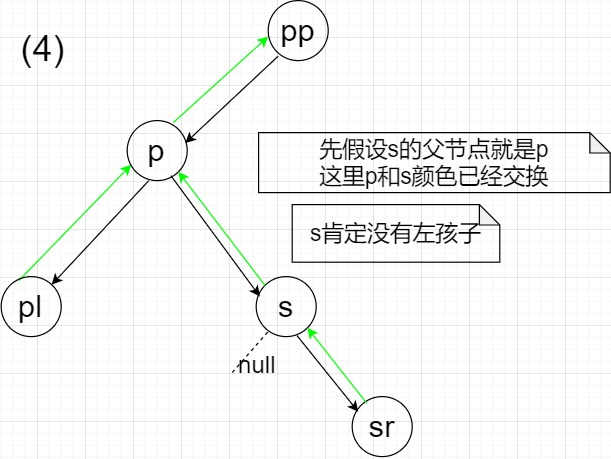• 执行完if ((s.left = pl) != null) pl.parent = s这句后，过程$(4)$会变成过程$(9)$，此时p和s已经交换了位置，也交换了颜色。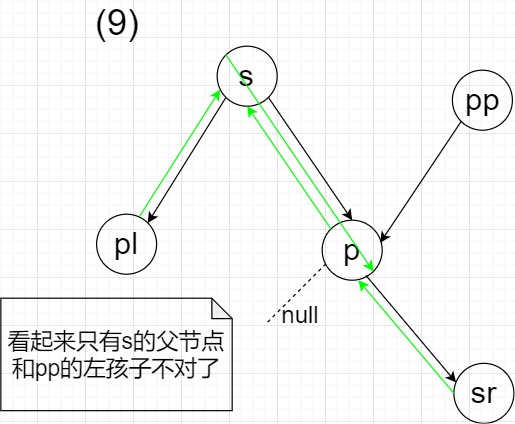• 根据之前的假设“p为pp左孩子”，所以过程$(9)$会转换到过程$(11)$（包括if ((s.parent = pp) == null)在内的三个分支），且会使得s与pp之间保持之前p与pp相同的孩子（之前p为pp左孩子，现在s为pp左孩子）。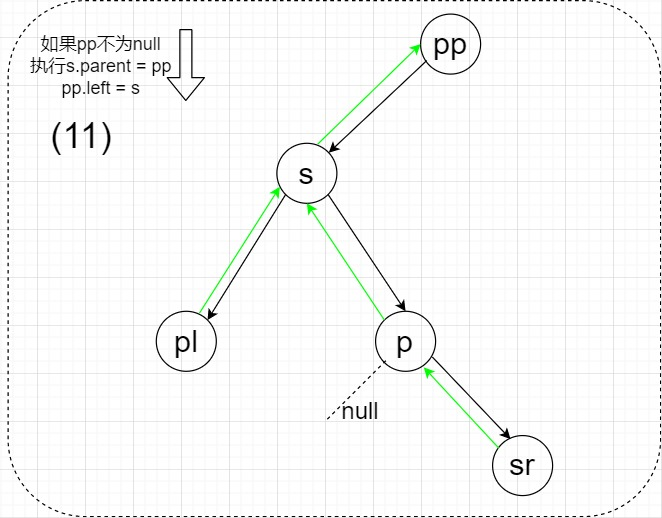• 过程$(11)$会根据sr是否为null来判断分支（包括if (sr != null)在内的两个分支），分别执行replacement = sr或者replacement = p。根据之前的讲解，可以得到，前者replacement = sr代表会在balanceDeletion函数调用前执行红黑树视图的删除操作（调用前会进入if (replacement != p)分支，调用后不会进入if (replacement == p)分支）；后者replacement = p代表会在balanceDeletion函数调用后执行红黑树视图的删除操作（调用前不会进入if (replacement != p)分支，调用后会进入if (replacement == p)分支）。
• 之所以上条分析跟之前的十分类似，是因为现在过程$(11)$只要之后删除掉p节点再使得s和sr相连，在不考虑平衡的情况下，删除操作就可以完成了。而如果sr为null，那其实就类似之前的“第一种情况：pl和pr都为null”，而如果sr不为null，那其实就类似之前的“第二种情况或第三种情况：pl和pr有一个null”。
• 过程$(11)$会根据sr是否为null来判断分支，分别走$(17)-(20)$$(21)-(22)$。如下图，观察两种情况下的最终结果。过程$(22)$中虽然p还没有脱离红黑树，但之后的if (replacement == p)分支会使得其脱离。这句TreeNode<K,V> r = p.red ? root : balanceDeletion(root, replacement)将会在最终结果后执行，p如果为红色，则不用调用balanceDeletion函数了，但p为红色代表初始时s为红色，之前讲过删除掉删除节点p相当于删除掉替换节点s，既然删掉的s为红色，那说明肯定不会平衡造成影响，所以不用调用balanceDeletion函数。而如果p为黑色，则需要调用balanceDeletion函数了。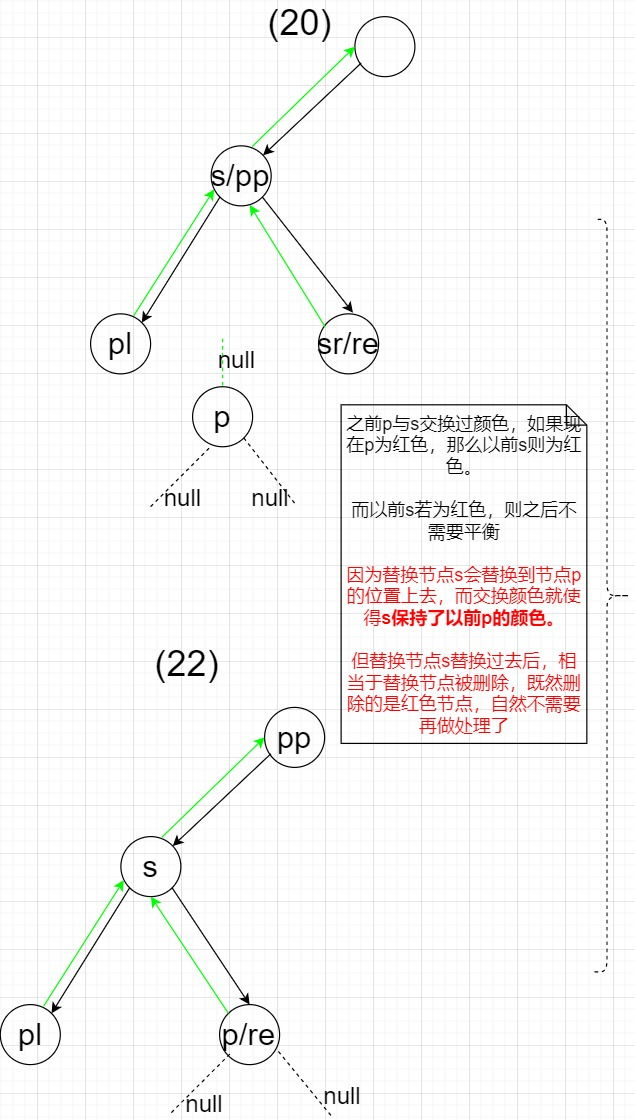• 分析下$(17)-(20)$$(21)-(22)$的开始状态和结束状态，如下图所示。可以发现，两种情况下都是，当调用balanceDeletion时，replacement节点子树的黑节点数肯定比它的兄弟节点的黑节点数少1了 。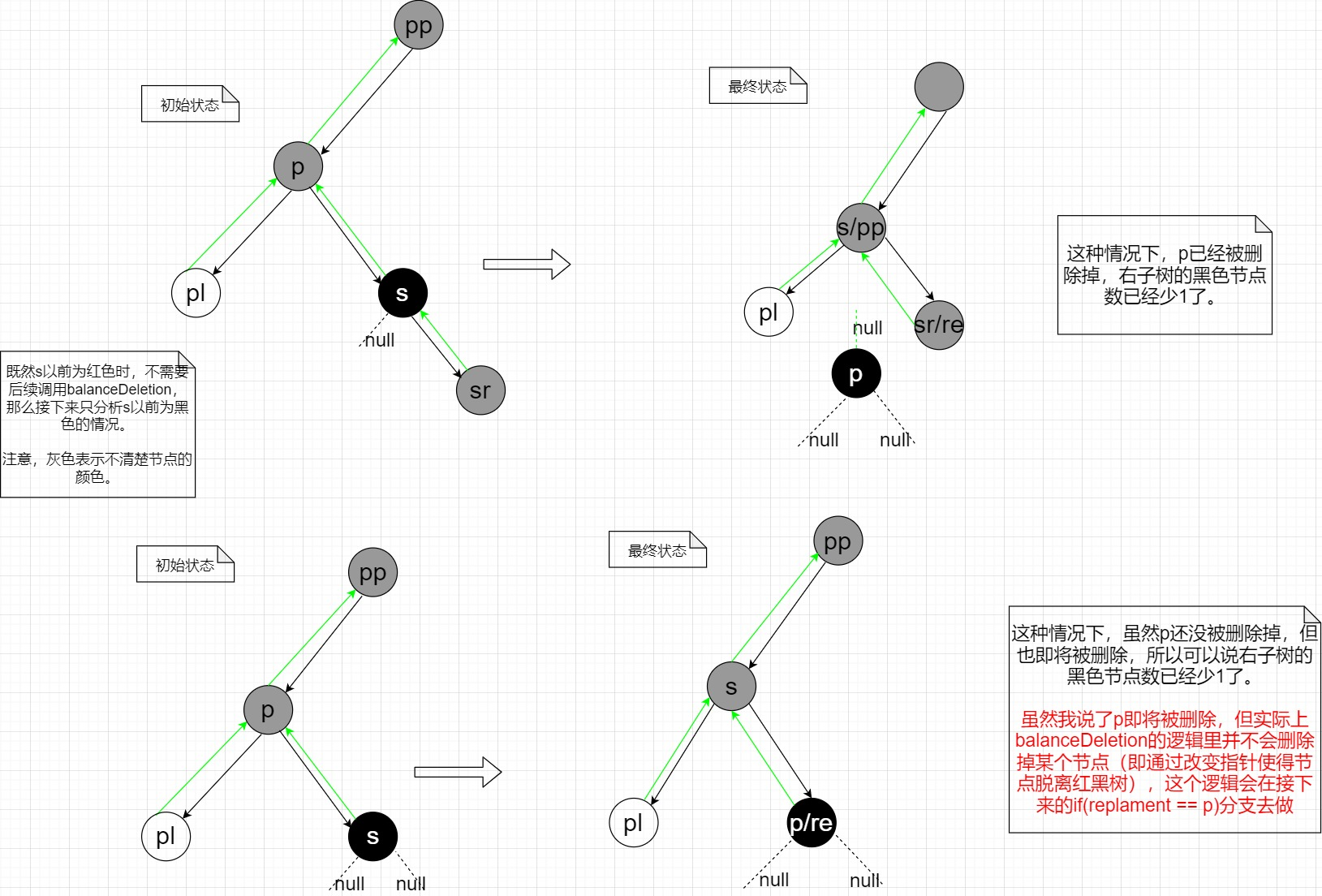• 而且上图的第二行的最终状态，说明了调用balanceDeletion函数后，s和p/re节点之间还是会保持父子关系的。这一点读者可以从balanceDeletion函数的大流程图中看出来，进入balanceDeletion函数后，s和p/re节点则是xp和x节点了。
• 大图中，过程$(3)$的后续过程也就不需要画出了，因为执行if (pl != null && pr != null)分支的情况也就是把先把p和s交换位置，并交换颜色，而且会使得s保持之前的孩子关系。
• 单独说一下if (movable) moveRootToFront(tab, r);，由于HashMap源码里实参的保证，这个movable肯定为true。

• p是删除节点，s才是“重要观点”里的替换节点，而replacement只是一个与兄弟节点不平衡的一个需调整的节点。
• 此函数做的事情，只是为了将删除节点从双链表视图和红黑树视图中都给删除掉。但区别在于，删除操作要么此句TreeNode<K,V> r = p.red ? root : balanceDeletion(root, replacement)之前做（指if (replacement != p)分支，说明实际被删节点有一个孩子，强调“实际被删”是因为重要观点里说了有时候替换节点相当于被删除），要么在之后做（指if (replacement == p)分支，说明实际被删节点没有孩子）。

# split

split函数只会在resize里面被调用到，观察与split调用处平级的链表操作逻辑，发现链表操作逻辑只是做了一个链表分离的过程（哈希桶内各节点，要么还在原哈希桶里，要么就在新哈希桶里），带着这一理解，我们开始分析。

        final void split(HashMap<K,V> map, Node<K,V>[] tab, int index, int bit) {//bit变量是那个重要bit为1时的int值。是resize的旧容量
//刚开始时，this为要分离的那个哈希桶的双链表视图下的第一个节点，因为this是对table取下标而来的
TreeNode<K,V> b = this;
// Relink into lo and hi lists, preserving order
int lc = 0, hc = 0;//统计low和high两个的节点数量
for (TreeNode<K,V> e = b, next; e != null; e = next) {//循环最后e=next使得e往next方向移动
//e为当前处理节点
next = (TreeNode<K,V>)e.next;//保持next的引用
e.next = null;//清空e的next成员
if ((e.hash & bit) == 0) {//那个重要bit为0，说明e应该在原哈希桶low里
if ((e.prev = loTail) == null)//将e与tail通过e.prev相连;如果low里还没有节点，那么新节点则作为head。
else
loTail.next = e;
loTail = e;
++lc;
}
else {//那个重要bit为1，说明e应该在新哈希桶high里
if ((e.prev = hiTail) == null)
else
hiTail.next = e;
hiTail = e;
++hc;
}
}

if (lc <= UNTREEIFY_THRESHOLD)//如果小于阈值，则不需要维持红黑树结构了
else {
if (hiHead != null) // 如果high里也有节点，说明low和high二者的红黑树结构由于拆分都应该被破坏掉了，所以需要树化
//反之，如果high里没有节点，那说明所有节点都在low里面。又由于连接链表时是按照原顺序来的，而原顺序又保持了
//红黑树结构（前提节点都在一个桶里）。所以就不需要做树化操作了，因为红黑树结构没有破坏。
}
}
if (hc <= UNTREEIFY_THRESHOLD)
else {
}
}
}

• 关于传入参数bit，请去看本人博客JDK8 HashMap源码全解析里关于resize函数的讲解。
• 循环里，如果e应该在原哈希桶low里，会进入到if ((e.hash & bit) == 0)分支。里面的逻辑分为两种情况（if ((e.prev = loTail) == null)分支和它的else分支）：如果low里一个节点都还没有，那么会进入前者，会执行到e.prev = loTail，此时low里有第一个节点了，且e的prev和next都为null，然后再更新head和tail；如果low里已经有节点了，那么会进入后者，会执行到e.prev = loTailloTail.next = e，这样就使得e和tail在双链表视图下相连，然后再只更新tail。
• 循环里，如果e应该在原哈希桶high里，分析同上。
• if (loHead != null)if (hiHead != null)分支里，在count值大于阈值有时候不需要进行树化以重塑由于拆分而被破坏掉的红黑树结构。这个特殊情况是因为所有节点都在原哈希桶low里，或新哈希桶high里，你想，执行此函数前，原哈希桶已经是红黑树结构了，现在我只是按照原来的顺序把它们又组装起来，组装完后，肯定还是完整的红黑树结构啊。

# rotateLeft

        static <K,V> TreeNode<K,V> rotateLeft(TreeNode<K,V> root,
TreeNode<K,V> p) {
TreeNode<K,V> r, pp, rl;
if (p != null && (r = p.right) != null) {
if ((rl = p.right = r.left) != null)
rl.parent = p;
if ((pp = r.parent = p.parent) == null)
(root = r).red = false;
else if (pp.left == p)
pp.left = r;
else
pp.right = r;
r.left = p;
p.parent = r;
}
return root;
}

• 初始状态下：rp的right child、ppp的parent、rlr的left child。
• p为rotateLeft函数要处理那个的节点。作为此函数的入参，一般认为p必有一个right child，即认为if (p != null && (r = p.right) != null)分支一定能进入。
• if ((rl = p.right = r.left) != null) rl.parent = p;中，我们先认为r.left肯定不为null（其实无论它为不为null对旋转结果都没有影响，后面会讲到），那么把这一句拆成rl = r.leftp.right = rl，其过程如下图所示。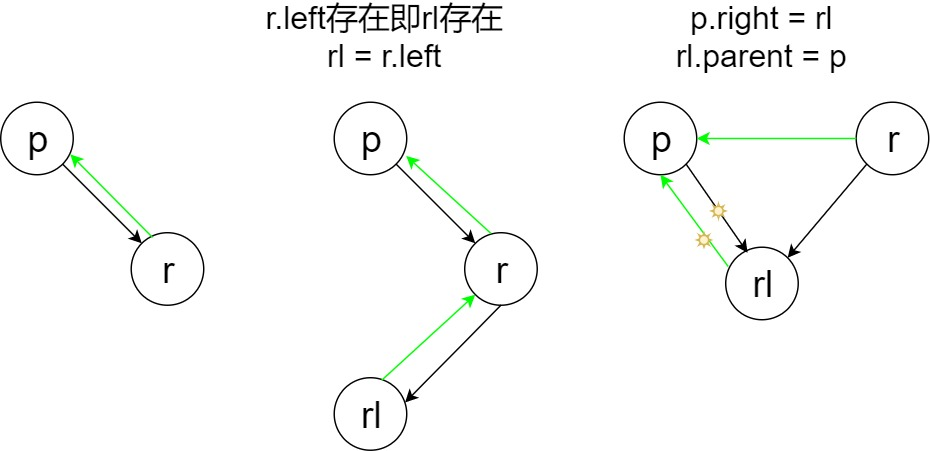• 示意图中，节点无颜色代表并不关心该节点的颜色，黑色箭头为左右孩子指针，绿色箭头为父亲指针。刚改变过指向的指针会用太阳标志标识出来。
• 接下来的if else嵌套有三个分支，这里不按照代码顺序分析，先假设程序会进入else if (pp.left == p)分支，此时说明之前的if分支没有进入，即pp不为null，ppp的左孩子。这里我们把pp = r.parent = p.parent拆分为pp = p.parentr.parent = pp。这样，从r.parent = pp开始执行到最后的示意图如下：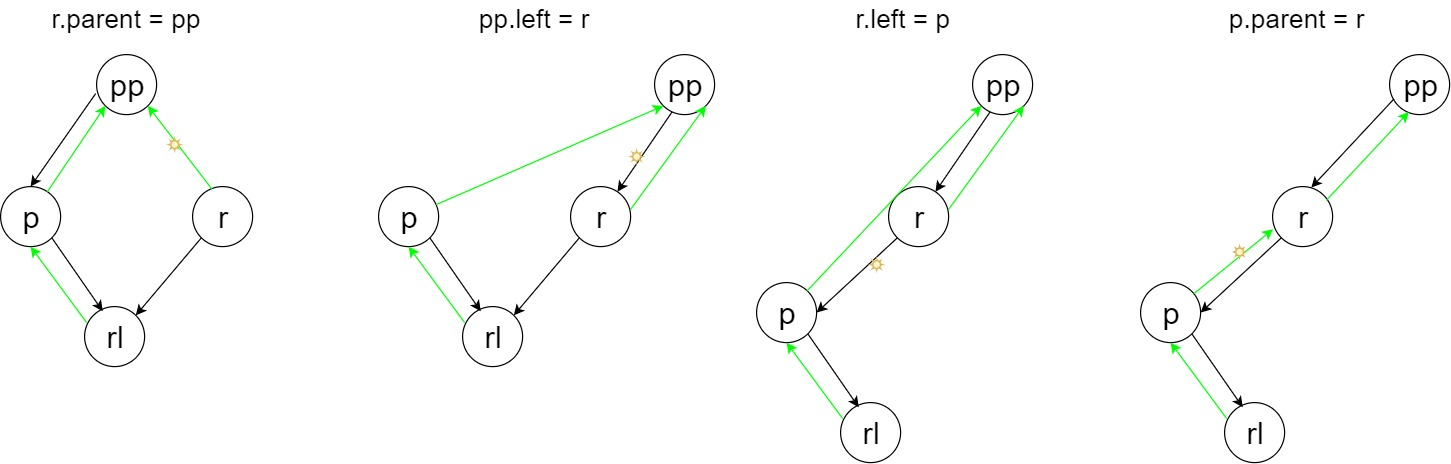• 再假设程序会进入最后的else分支，说明pp不为null，ppp的右孩子。同样的，我们把pp = r.parent = p.parent拆分为pp = p.parentr.parent = pp。这样，从r.parent = pp开始执行到最后的示意图如下：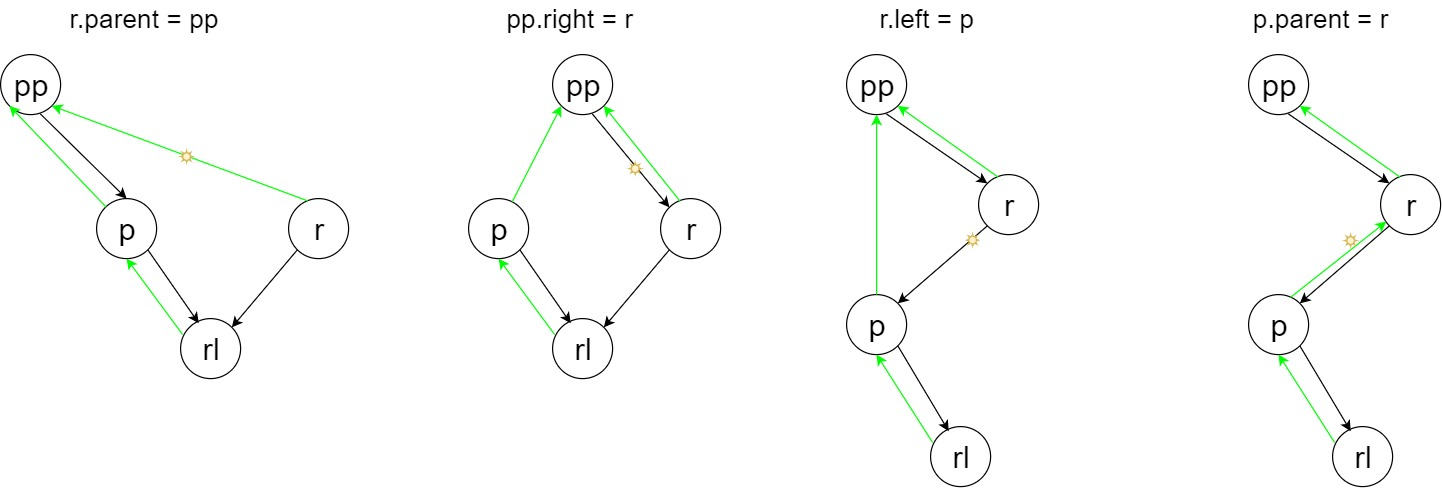• 最后再假设程序会进入if ((pp = r.parent = p.parent) == null)分支，说明pp为null。同样的，我们把pp = r.parent = p.parent拆分为pp = p.parentr.parent = pp。但进入这个分支说明pp为null，这样，从r.parent = pp（实际是r.parent = null）开始执行到最后的示意图如下：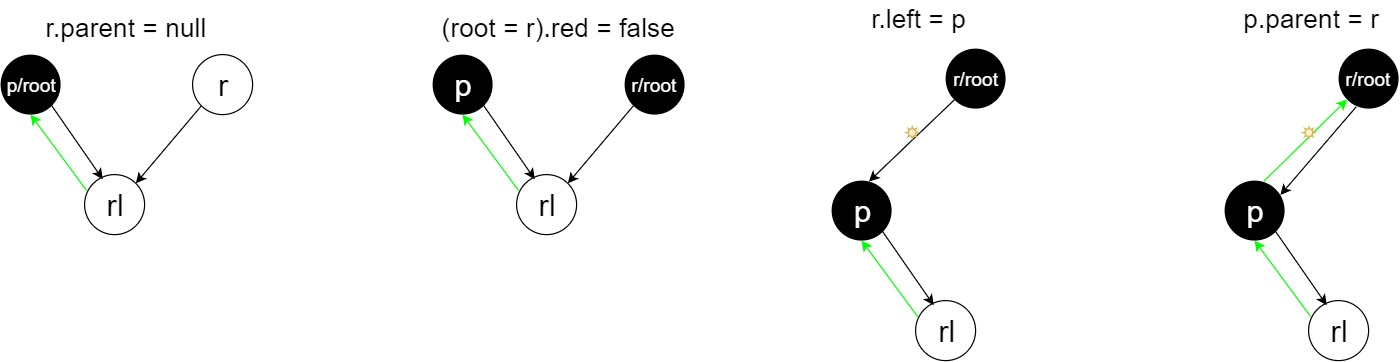• 此函数并不关心旋转后红黑树是否平衡，它只负责完成旋转的任务，所以，是此函数的调用者负责维持平衡。
• 此函数的完整流程示意图如下。将三种情况对比分析后，可以发现，第4步和第5步都是为了处理好ppr之间的连接，pp作为p的父节点，是整个旋转部分的上层，旋转后pp还是会与下层保持相同的孩子关系（原来ppp的什么孩子，现在r就会是pp的什么孩子）（第三种情况由于pp为null，所以就不用处理ppr之间的连接）。
• 第6步和第7步都是为了完成旋转的后半部分，即处理好pr之间的连接，让p成为r的左孩子，完成左旋的任务。由于之前（第4、5步）已经处理好了p的父节点pp的孩子关系，所以可以改变p.parent了（反过来想，如果先执行第6、7步再执行第4、5步会导致pp节点再也找不到了，因为第7步会改变p.parent）。
• 第3步都是为了完成旋转的前半部分，即处理好prl之间的连接，让rl成为p的右孩子。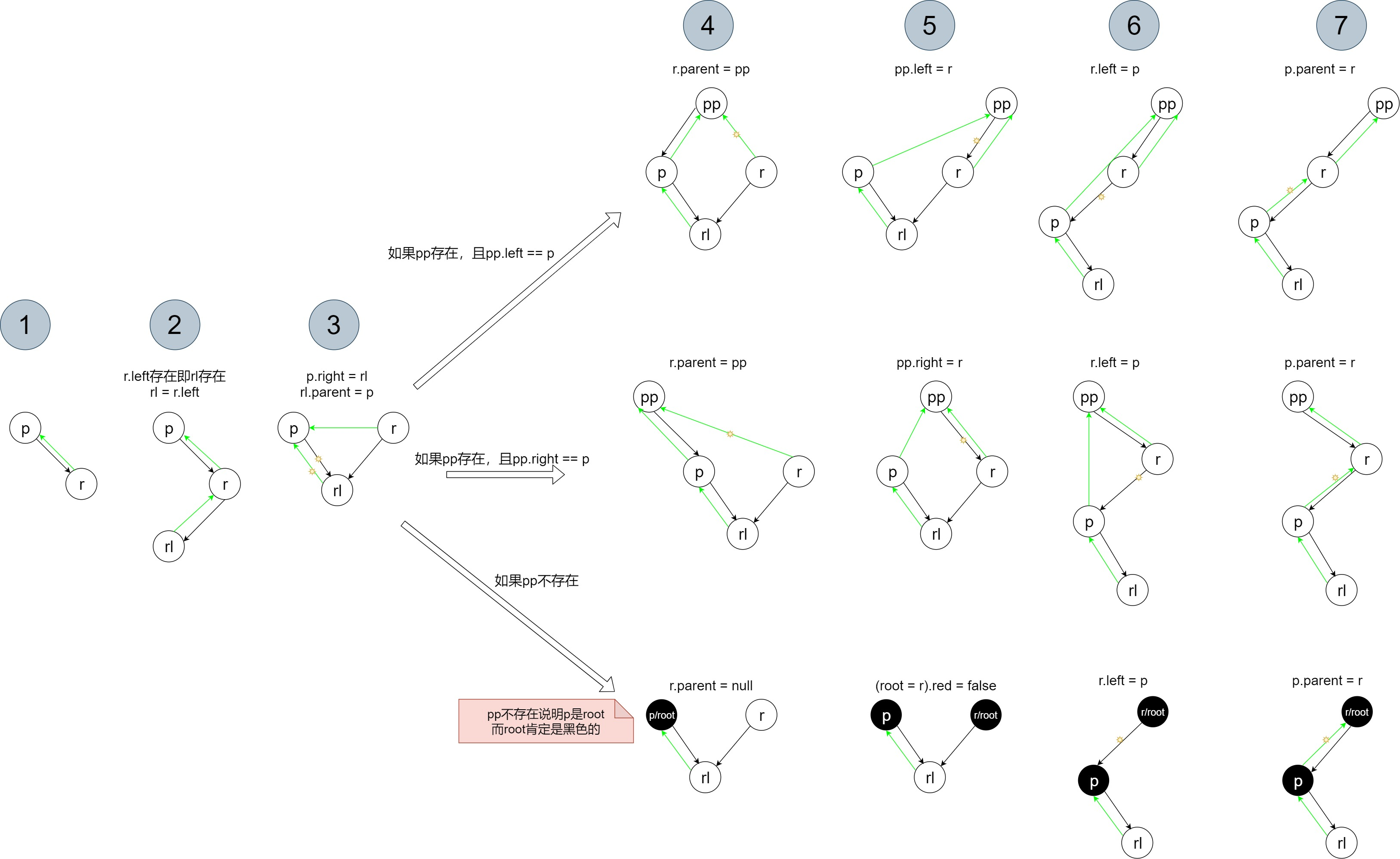• 若将最终旋转的结果总结一下，再忽略掉pp节点（因为pp节点其实不属于旋转部分，它只是等旋转好了以后再与新的旋转部分维持相同的孩子关系），可得出如下示意图。可以发现这种旋转十分巧妙，旋转后p节点的左孩子不会受到影响、r节点的右孩子不会受到影响、rl节点的左右孩子都不会受到影响。
• r.left是否存在，对旋转结果也不会产生本质影响。它只是会让p节点的右孩子为null。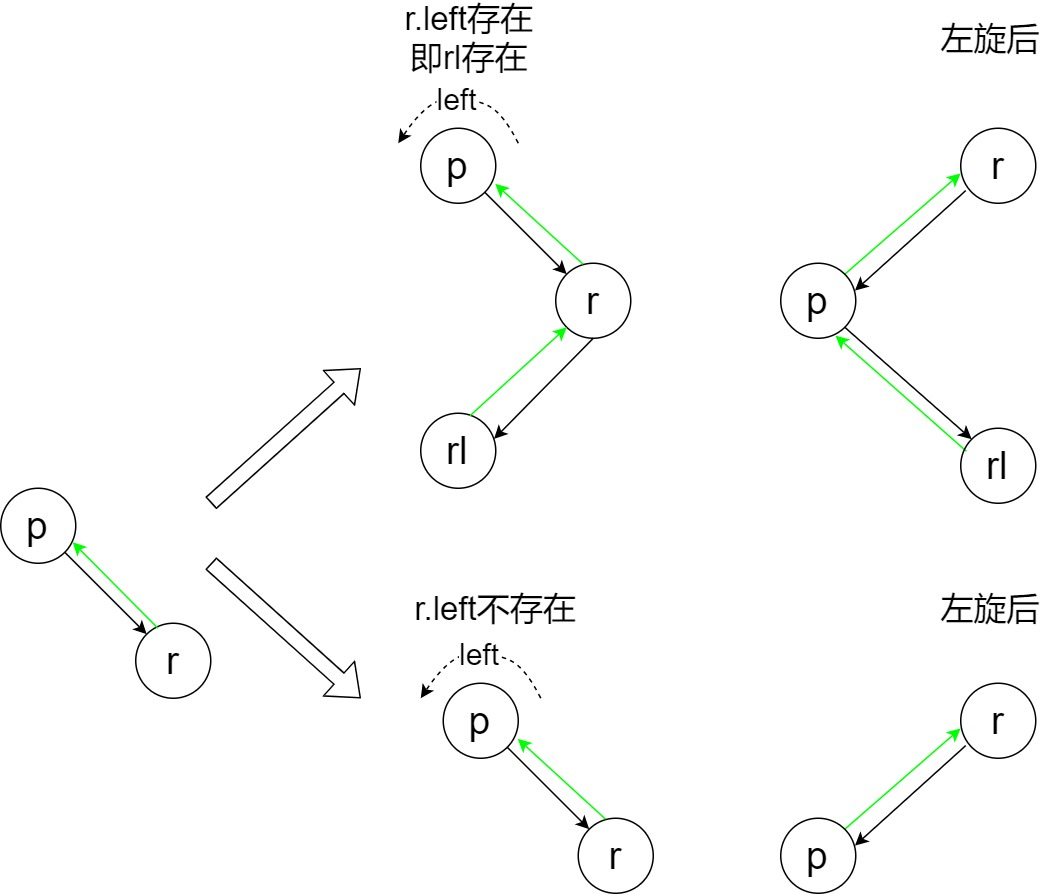# rotateRight

        static <K,V> TreeNode<K,V> rotateRight(TreeNode<K,V> root,
TreeNode<K,V> p) {
TreeNode<K,V> l, pp, lr;
if (p != null && (l = p.left) != null) {
if ((lr = p.left = l.right) != null)
lr.parent = p;
if ((pp = l.parent = p.parent) == null)
(root = l).red = false;
else if (pp.right == p)
pp.right = l;
else
pp.left = l;
l.right = p;
p.parent = l;
}
return root;
}

• 初始状态下：lp的left child、ppp的parent、lrl的right child。
• 由于右旋和左旋完全类似，分析过程完全和上面章节类似，所以接下来只做重要讲解。
• if ((lr = p.left = l.right) != null)分支：lr = p.left = l.right拆分为lr = l.rightp.left = lr，然后接下来执行lr.parent = p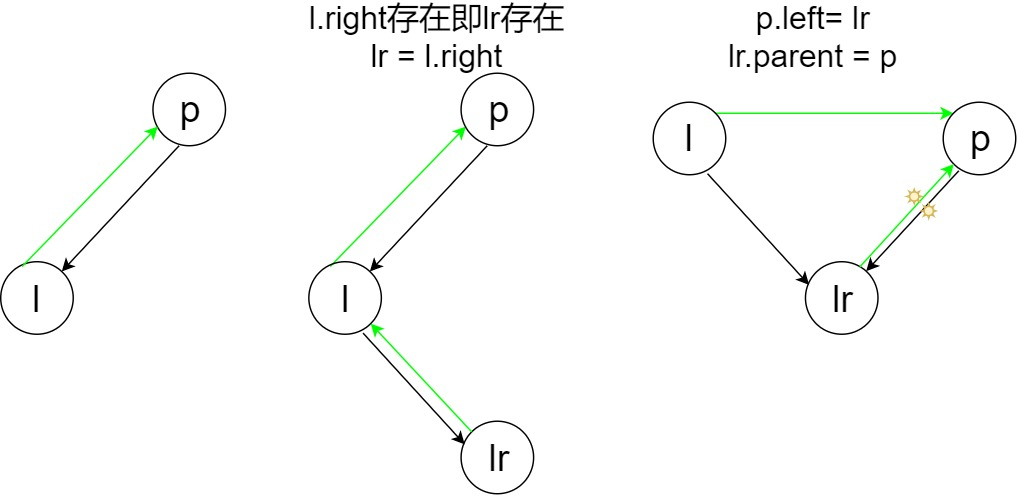• pp = l.parent = p.parent拆分为pp = p.parentl.parent = pp
• 先假设进入最后的else分支（代表pp.left == p成立），示意图如下：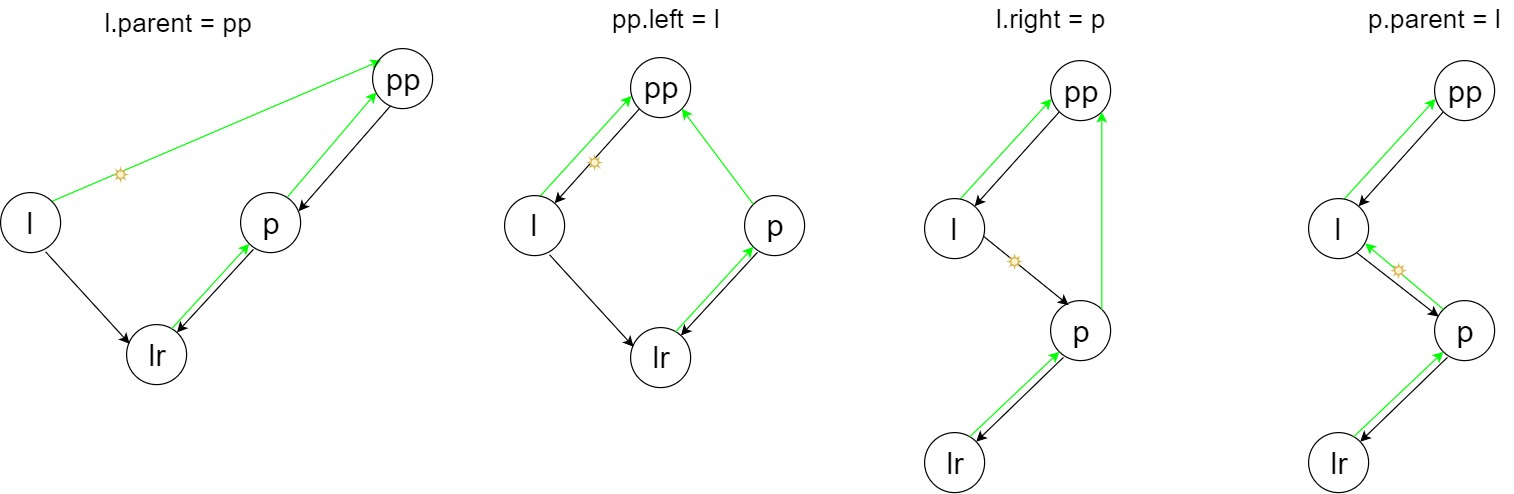• 再假设进入else if (pp.right == p)分支，示意图如下：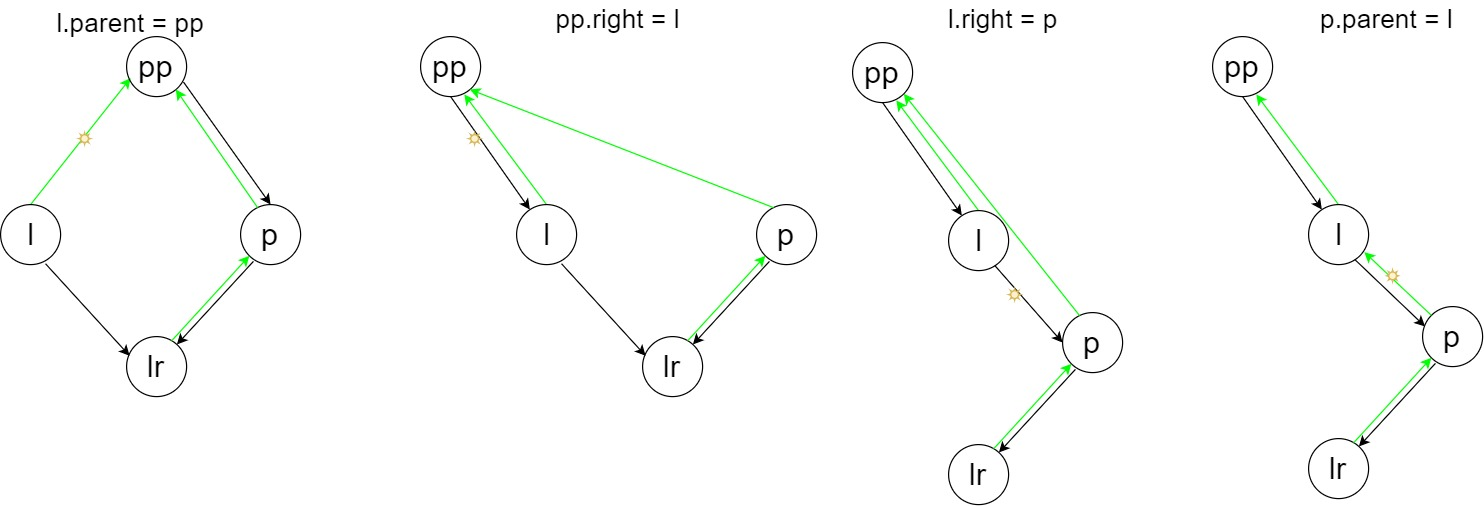• 最后假设进入if ((pp = l.parent = p.parent) == null)分支，示意图如下：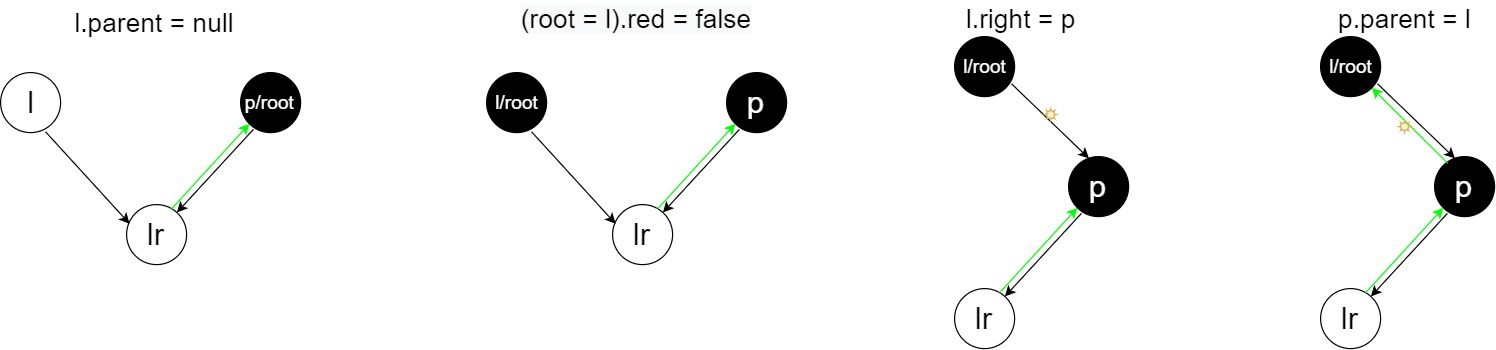• 完整流程示意图：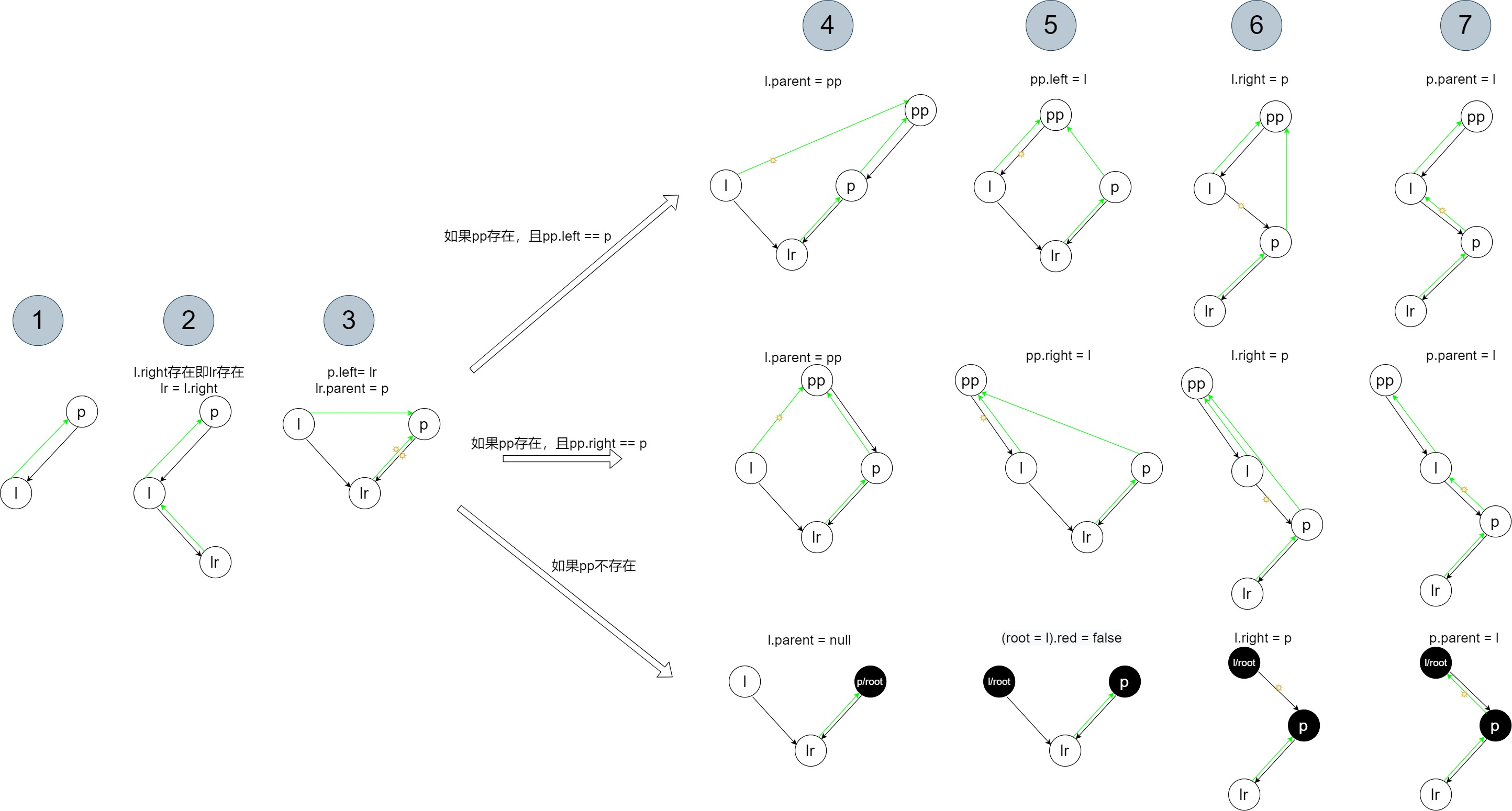• 右旋总结示意图：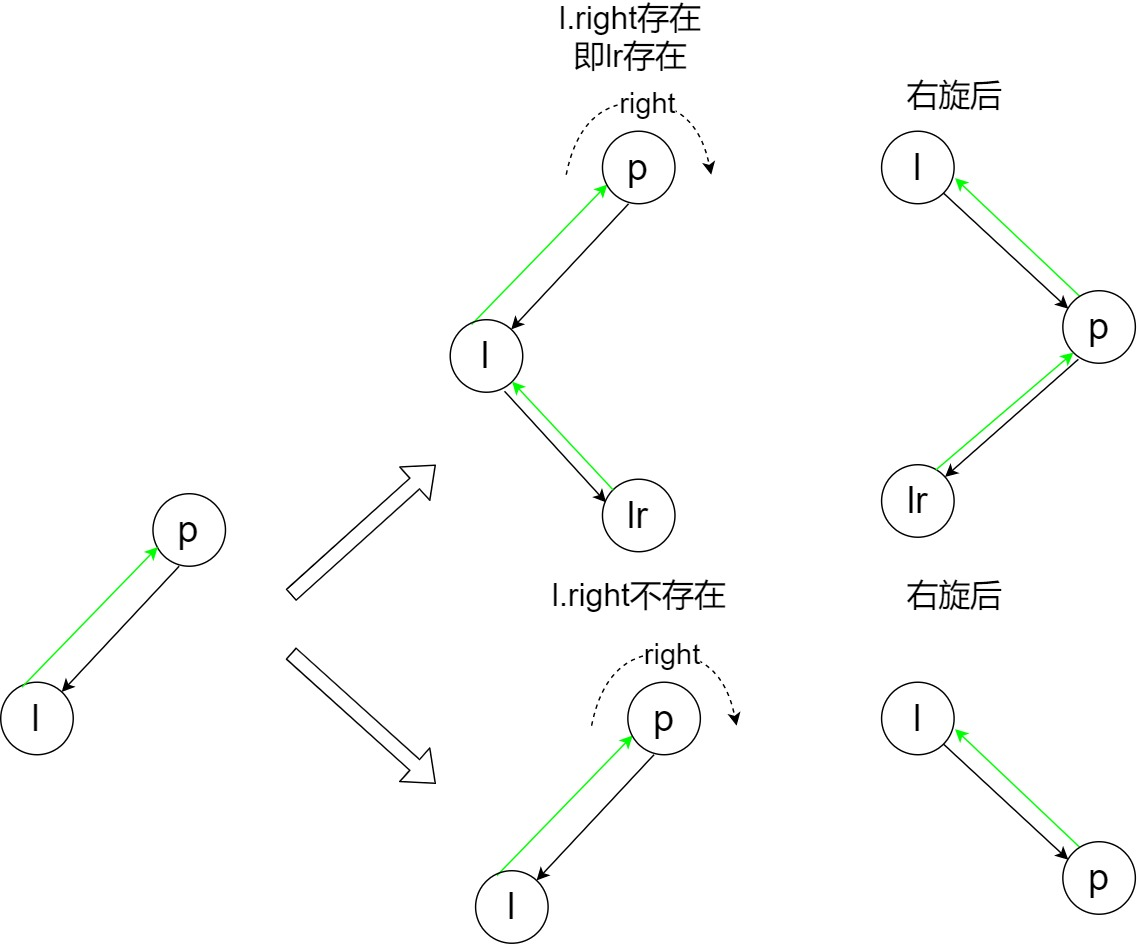# 左旋右旋总结

• 不管是左旋还是右旋，pp节点其实都不算是旋转的部分，因为在旋转后，它只是与新的旋转部分保持相同的孩子关系。
• 从左旋、右旋的总结示意图里可以看出，没有画出来的子树部分之所以不用画，是因为在旋转后子树会保持相同的相对位置。比如，左旋总结示意图中：p的左子树还会是p的左子树，r的右子树还会是r的右子树。右旋总结示意图中：p的右子树还会是p的右子树，l的左子树还会是l的左子树。

# balanceInsertion

static <K,V> TreeNode<K,V> balanceInsertion(TreeNode<K,V> root,
TreeNode<K,V> x) {
// 让新放置进来的节点为红色
x.red = true;
// xp父亲，xpp爷爷，xppl爷爷的左孩子，xppr爷爷的右孩子。xppl和xppr其中一个必和xp一样
for (TreeNode<K,V> xp, xpp, xppl, xppr;;) {
if ((xp = x.parent) == null) {//说明x是root。需留意何处调用此函数时，形参x会是根节点
x.red = false;
return x;//直接返回，不需调整
}
//如果父亲节点是黑的，或者
//父亲节点虽然是红色的，但爷爷节点为null。存疑，这种情况何时出现？
else if (!xp.red || (xpp = xp.parent) == null)
return root;
// 执行到这里，说明x的父亲xp是红色的。如果是第一次进入循环，那么x自己也是红色的，
// 至于第二次(或第n次)进入循环，x是不是红色的，得看下面的分析
// 如果父亲是爷爷的左孩子
if (xp == (xppl = xpp.left)) {
if ((xppr = xpp.right) != null && xppr.red) {//爷爷的右孩子不为null，且为红色
xppr.red = false;
xp.red = false;
xpp.red = true;
x = xpp; //让x指向爷爷节点，作为新x继续下一次循环，这里保证了新x是红色的
}
else {//要么是爷爷的右孩子为null，或者爷爷的右孩子为黑色
if (x == xp.right) {//如果x是xp的右孩子
root = rotateLeft(root, x = xp); //x指向了父亲节点
xpp = (xp = x.parent) == null ? null : xp.parent;//这里不用判断x是否为null吧？也用赋值xpp吧，左旋会处理父子关系的
}
//执行到这里，说明x是xp的左孩子了。有两种情况：1.没有执行上面if，本来x就是xp的左孩子
//2.本来x就是xp的右孩子，但执行了上面if后，被纠正过来了
if (xp != null) {
xp.red = false;
if (xpp != null) {
xpp.red = true;
root = rotateRight(root, xpp);
}
}
//执行完这两个if(也可能只执行了一个)后，下一次循环必然会进入循环终点之一else if (!xp.red || (xpp = xp.parent) == null)
//因为父亲节点是黑色。注意，这里保证了新x是红色的
}
}
// 如果父亲是爷爷的右孩子
else {
if (xppl != null && xppl.red) {//爷爷的左孩子不为null，且为红色
xppl.red = false;
xp.red = false;
xpp.red = true;
x = xpp; //让x指向爷爷节点，作为新x继续下一次循环，这里保证了新x是红色的
}
else { //要么是爷爷的左孩子为null，或者爷爷的左孩子为黑色
if (x == xp.left) {//如果x是xp的左孩子
root = rotateRight(root, x = xp);
xpp = (xp = x.parent) == null ? null : xp.parent;
}
//执行到这里，说明x是xp的右孩子了。有两种情况：1.没有执行上面if，本来x就是xp的右孩子
//2.本来x就是xp的左孩子，但执行了上面if后，被纠正过来了
if (xp != null) {
xp.red = false;
if (xpp != null) {
xpp.red = true;
root = rotateLeft(root, xpp);
}
}
//执行完这两个if(也可能只执行了一个)后，下一次循环必然会进入循环终点之一else if (!xp.red || (xpp = xp.parent) == null)
//因为父亲节点是黑色。注意，这里保证了新x是红色的
}
}
}
}

• 在新插入节点通过二叉查找的方式找到初始放置位置后，会调用此函数。
• 认为新插入节点的颜色是红色（第一行代码x.red = true），这样子是有好处，因为如果初始放置后，发现其父节点就是黑色的，就可以直接返回了（这样不会影响各个路径上的黑节点个数）。
• 主要逻辑是个循环过程，注意该循环并没有设置停止条件，只是在里面有两个break可以退出（搞得跟递归似的，终点设置在开头，过程设置在后面）。所以，其过程是循环一次或多次后到达了break的终点，然后退出循环。每次循环完成后，代表已经处理好x节点的左右平衡了，并且各路径的黑节点个数保持不变，但x引用可能会指向新的节点了。退出循环时，说明到达了循环的终点，且整棵树都已经调整平衡了。
• if ((xp = x.parent) == null)分支是终点之一，说明多次循环后，x已经到达了root节点。
• else if (!xp.red || (xpp = xp.parent) == null)分支也是终点之一，有两种情况：1.xp是黑色的，此时不管xp.parent == null是否成立，都是退出循环。 2.虽然xp是红色的，但如果xp.parent == null成立，退出循环。（个人感觉第二种情况不会进入的：第一点，此时xp为root，而root是不应该为红色的；第二点，每次循环结束后或者说每次循环开始时，x都是红色的，而xp也是红色的，这也不合理。）
• 如果两个终点都没有进入，那么说明xp是红色的，且xp.parent存在。这样，再算上x，那么有三层树的结构了，再对这三层树进行处理。

• 第一次进入循环时，x节点是红色的（第一行代码x.red = true）。我们先进行一个合理的假设：每次循环结束后（也是每次循环开始前），x节点也会是红色的。当分析完循环的所有分支时，你会发现这个假设成立。
• if (xp == (xppl = xpp.left))分支代表父亲是爷爷的左孩子，即xpp.left == xp成立，再往下看，又有一对if else。
• if ((xppr = xpp.right) != null && xppr.red)分支，代表爷爷的右孩子不为null，且为红色。示意图如下。因为xxp都是红色的，所以需要调整。发现完成后，左右两条路径的黑色节点数量相同，且保持了不变（数量还是1，如果我们认为底下的子树没有黑色节点了）。这样的好处是，如果xpp有一个兄弟节点，并不会造成xpp与其兄弟节点之间的不平衡。循环结束后，x引用指向了新的节点，且为红色。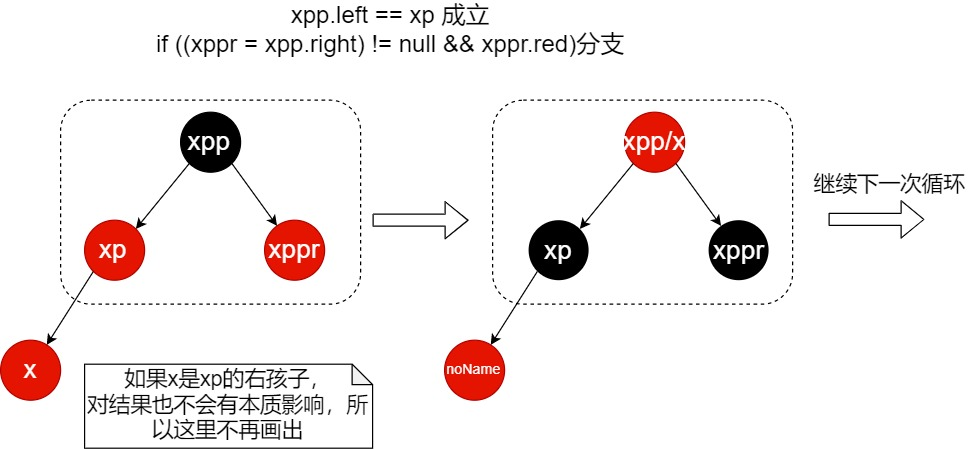• if ((xppr = xpp.right) != null && xppr.red)的else分支里有两个if，但第一个if不一定会执行。所以有两种情况，首先分析两个if都进入的情况。示意图如下（注意图中有两个节点标注了1,2，因为xxp这两个引用会互换，所以必须看它们真实的标号；xppxppr中之间的虚线代表xppr要么不存在，要么为黑色）。最终结果看起来是把2号节点移到了上面去。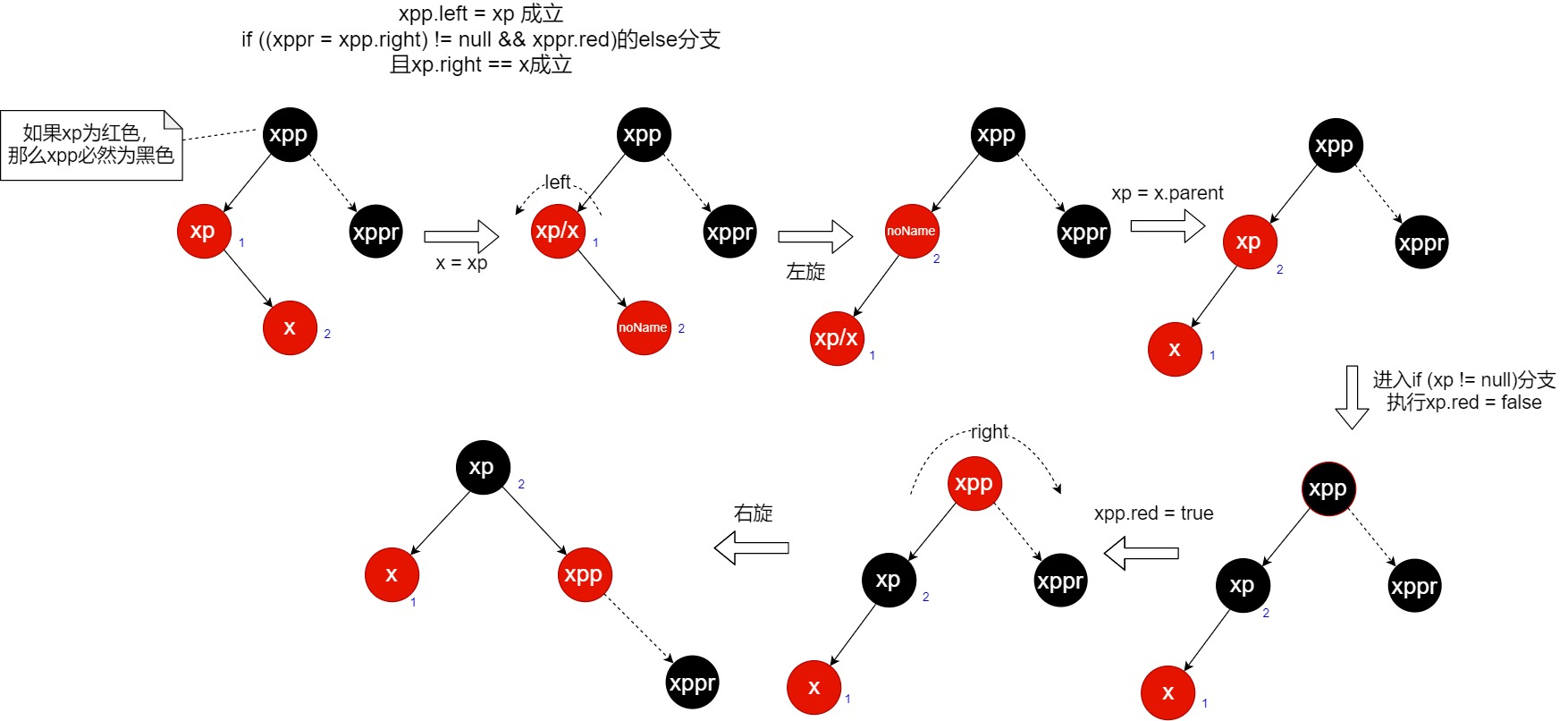• 这里开始论证为什么上图的最终状态是对的。将上图的开始状态和结束状态进行必须的补充，可以得到下图。联系之前说到的“每次循环完成后，代表已经处理好x节点的左右平衡了，并且各路径的黑节点个数保持不变”，所以开始状态时x节点是符合这句话的。从xpp往下看，xpp的各个路径的黑节点数也是相同的，由于xpp每条路径都有，那么去掉它，每条路径的黑节点数必定也是相同的，往右看，遇到了xppr，那么假设xppr这颗子树各路径的黑节点个数是n；往左看，遇到了xp，但由于它是红色的，不会对黑节点个数造成影响，所以看它的子树，所以TREE1这颗子树的黑节点个数也会是n；倒回来再遇到x，同理分析，那么TREE2TREE3也等于n。而到了结束状态，发现2号节点的原左子树TREE2成为了1号节点的右子树（因为左旋操作），2号节点的原右子树TREE3成为了xpp的右子树（因为右旋操作），再按同理分析，发现结束状态的各个路径的黑节点个数也是相同的。通过分析原子树与现子树，证毕。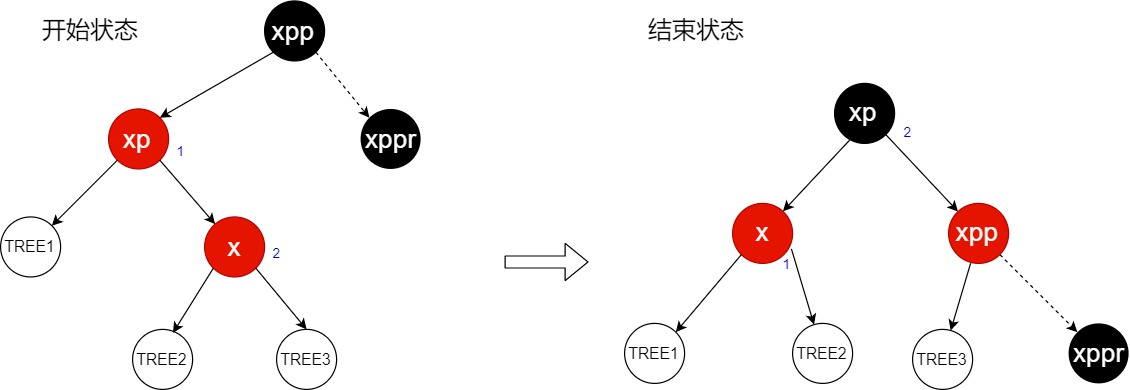• if ((xppr = xpp.right) != null && xppr.red)的else分支结束循环后，会进入第二个终点else if (!xp.red || (xpp = xp.parent) == null)
• 分析if ((xppr = xpp.right) != null && xppr.red)的else分支里的第二种情况，即只执行第二个if。示意图如下，同理分析原子树与现子树，也能证明下图的最终状态是平衡的。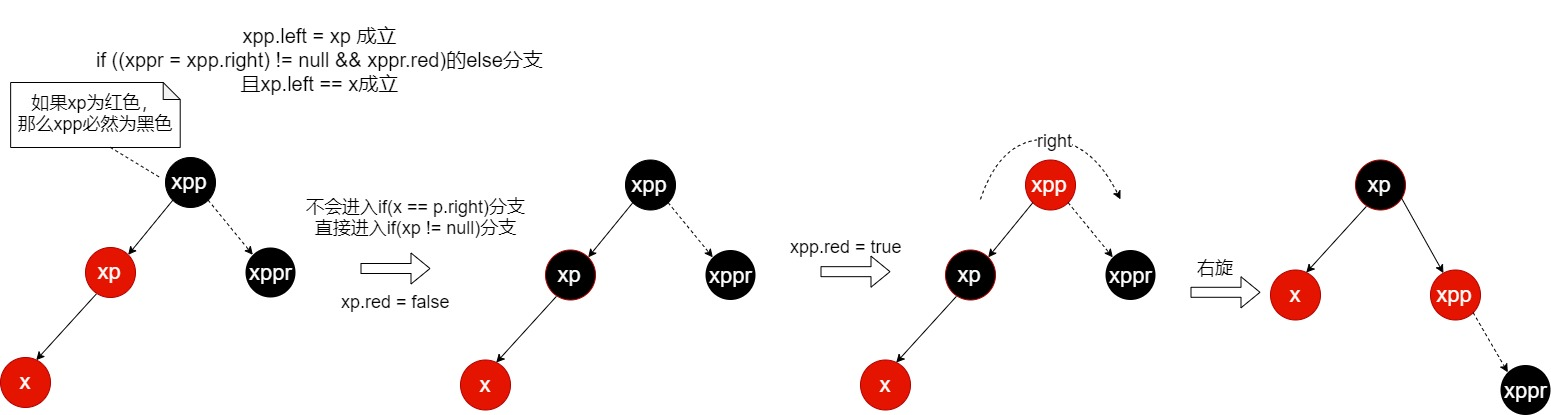• 其实这两个连续的if的第一个if就是为了调整xp与x的孩子关系，如果x是xp的右孩子，那么必须先调整成左孩子；如果x是xp的左孩子，那就不用调整。这里你可能想杠一下，如果就算x是xp的右孩子，我也只执行第二个if，会发生错误吗，是的，会发生。示意图如下，这样做了以后，会使得当前循环处理的三层树结构在循环结束后还存在着两个连续的红色节点。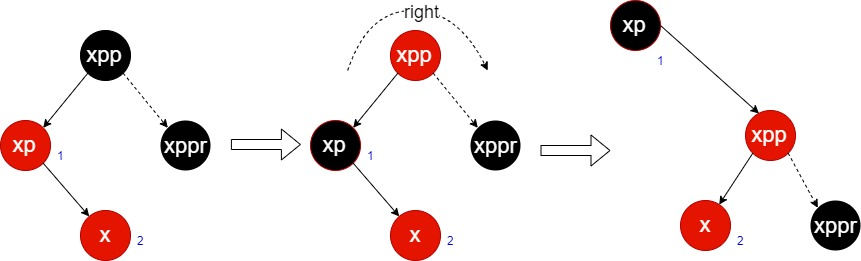if (xp == (xppl = xpp.left))的else分支代表父亲是爷爷的右孩子，即xpp.right == xp成立，接下来分析这里面的情况，但过程完全与上面类似：

• if (xppl != null && xppl.red)分支，示意图如下：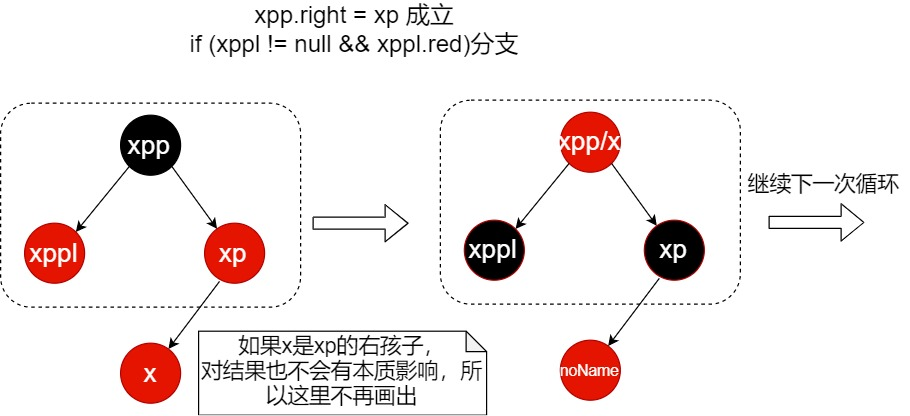• if (xppl != null && xppl.red)的else分支，如果里面两个if都走：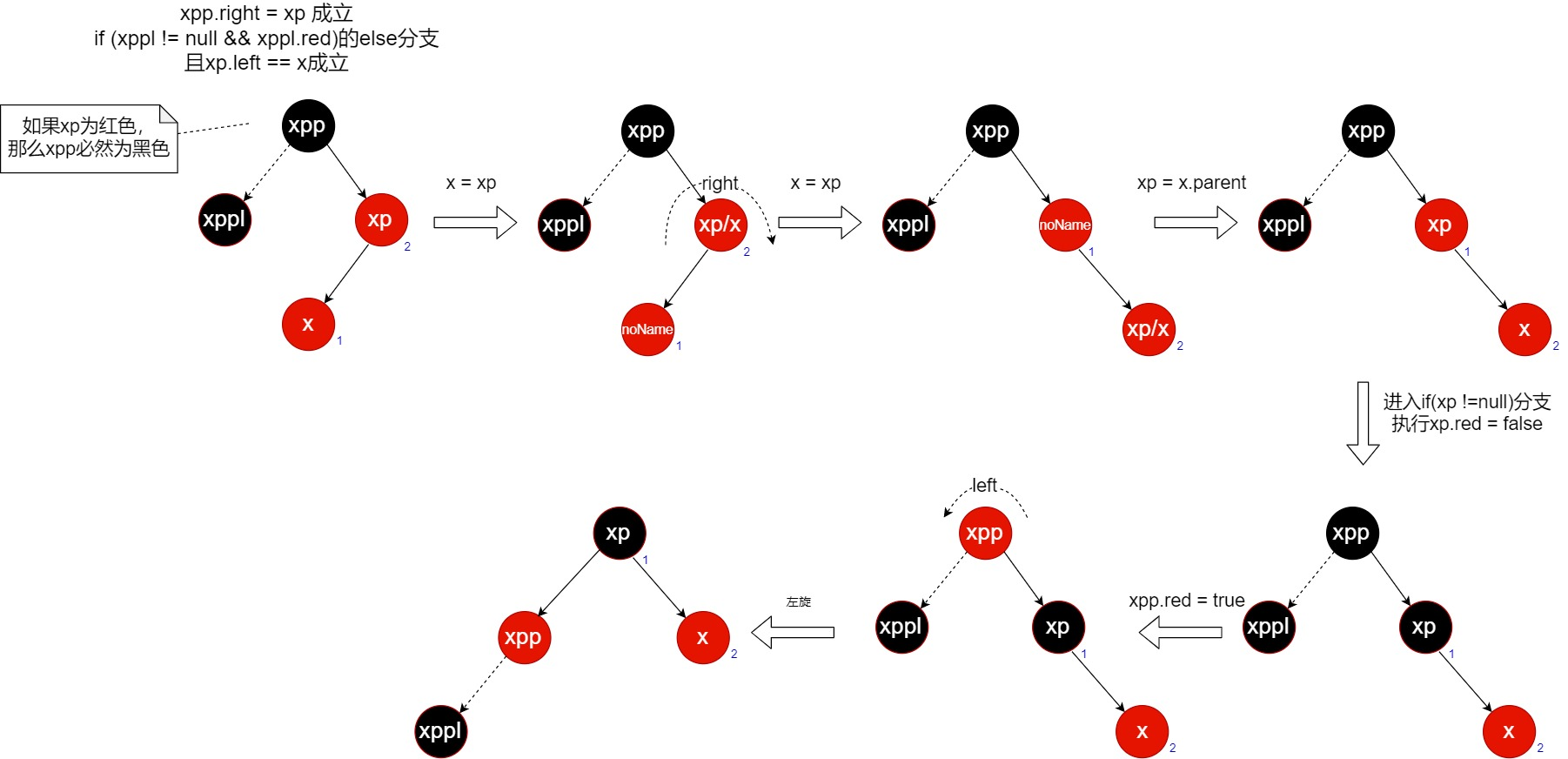• if (xppl != null && xppl.red)的else分支，如果里面只走第二个if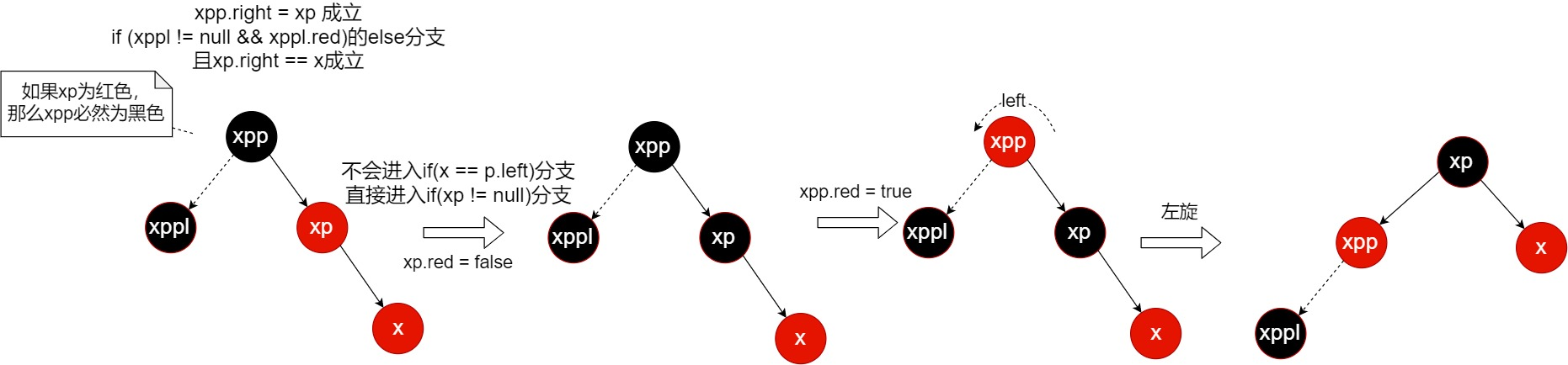• 每次循环都是处理三层树的结构，处理完毕时，这三层树结构的各个路径上的黑色节点个数相比之前会是一样的。虽然下一次循环开始时，各路径的黑色节点个数还是一样的，但却可能不再满足“相邻节点不能都是红色的”这一条件了，所以又需要继续处理。
• 每次循环结束后，三层树结构里不会再有连续的红节点。
• 循环的结束状态相比开始状态，x引用会往上移动，所以下一次循环开始时可能不再满足“相邻节点不能都是红色的”这一条件。
• 需要有一个大前提，就是循环开始，整棵树是平衡的。然后，各个循环才能环环相扣，每次循环结束时，保持各个路径上的黑色节点个数不变。

# balanceDeletion

        static <K,V> TreeNode<K,V> balanceDeletion(TreeNode<K,V> root,
TreeNode<K,V> x) {
//注意，传进来的x节点子树的黑节点数，肯定是比x的兄弟节点子树的黑节点数少1
for (TreeNode<K,V> xp, xpl, xpr;;)  {
if (x == null || x == root)//如果x是root
return root;
else if ((xp = x.parent) == null) {//（说明是循环后更新x后，使得x指向了root）但x没有父节点
x.red = false;
return x;
}
else if (x.red) {//如果x不是root(有父节点)，且x为红色（这好办，直接把x变成黑色，让x子树的黑节点+1.多次循环可到达此分支）
x.red = false;
return root;
}
//接下来两个分支，x必为黑色
else if ((xpl = xp.left) == x) {//如果x是xp的左孩子
if ((xpr = xp.right) != null && xpr.red) {
xpr.red = false;
xp.red = true;
root = rotateLeft(root, xp);
xpr = (xp = x.parent) == null ? null : xp.right;
}
if (xpr == null)
x = xp;
else {
TreeNode<K,V> sl = xpr.left, sr = xpr.right;
if ((sr == null || !sr.red) &&
(sl == null || !sl.red)) {
xpr.red = true;
x = xp;
}
else {
if (sr == null || !sr.red) {
if (sl != null)
sl.red = false;
xpr.red = true;
root = rotateRight(root, xpr);
xpr = (xp = x.parent) == null ?
null : xp.right;
}
if (xpr != null) {
xpr.red = (xp == null) ? false : xp.red;
if ((sr = xpr.right) != null)
sr.red = false;
}
if (xp != null) {
xp.red = false;
root = rotateLeft(root, xp);
}
x = root;
}
}
}
else { // symmetric//如果x是xp的右孩子
if (xpl != null && xpl.red) {
xpl.red = false;
xp.red = true;
root = rotateRight(root, xp);
xpl = (xp = x.parent) == null ? null : xp.left;
}
//经过上面if，不管它有没有执行，x的兄弟xpl肯定为黑色节点了
if (xpl == null)
x = xp;
else {
TreeNode<K,V> sl = xpl.left, sr = xpl.right;
if ((sl == null || !sl.red) &&//这种情况说明xpl的孩子里没有红色节点
(sr == null || !sr.red)) {
xpl.red = true;
x = xp;
}
else {//这种情况说明xpl的孩子里有红色节点
if (sl == null || !sl.red) {//如果sr为红色，则走此分支；sr其他情况则不会
if (sr != null)
sr.red = false;
xpl.red = true;
root = rotateLeft(root, xpl);
xpl = (xp = x.parent) == null ?
null : xp.left;
}
if (xpl != null) {
xpl.red = (xp == null) ? false : xp.red;//xpl最终会旋转到之前xp的位置，并保持xp的颜色
if ((sl = xpl.left) != null)
sl.red = false;
}
if (xp != null) {
xp.red = false;
root = rotateRight(root, xp);
}
x = root;//下一次循环直接返回
}
}
}
}
}

• 提前说明一下，当说到“x节点子树的黑节点数n”是指：从x节点到它的子树的任意一个叶子节点的路径上的黑色节点个数都等于n。
• 整个函数是一个循环过程，可能会经过若干次循环。不管是刚调用此函数的第一次循环，或者是以后的循环，每次循环体刚开始时，x节点子树的黑节点数，肯定是比x的兄弟节点子树的黑节点数少1，这是由removeTreeNode函数来做保证的（由于删掉了一个黑色节点，所以黑节点数少1）。既然知道了x的黑节点数，比x的兄弟节点饿黑节点数少1，那么就需要通过调整来使得平衡。
• if (x == null || x == root)分支，如果x是root，则直接返回root。上一次循环执行了x = root后，会进入此分支。
• else if ((xp = x.parent) == null)分支，x的父节点xp为null，但xp为null说明x为root，但这样的话则只会进入上面的if (x == null || x == root)分支了，所以我认为此分支不可能进入。
• else if (x.red)分支，说明x不是root节点，且x为红色。这好办，直接把x变成黑色，让x的黑节点数+1。这样x的黑节点数就和x的兄弟节点的黑节点数一样了，也就到达了平衡。
• 接下来的两个分支，说明x不是root节点，且x为黑色，所以调整过程要稍微复杂一点了。但这两个分支是完全对称的，所以我只会讲一个分支。由于removeTreeNode函数的保证（总是以删除节点的后继作为替换节点，这里后继是指刚好大于删除节点的那个节点），所以调用此函数时，x肯定是xp的右孩子，所以我接下来讲解else if ((xpl = xp.left) == x)的else分支。
• 接下来这个大图是整个函数的else if ((xpl = xp.left) == x)的else分支的所有过程，每个过程都有标号以方便讲解。节点除标明为黑色或者红色外，灰色则代表不清楚此节点的颜色。建议读者对照着大图、源码和本博客同时进行查阅。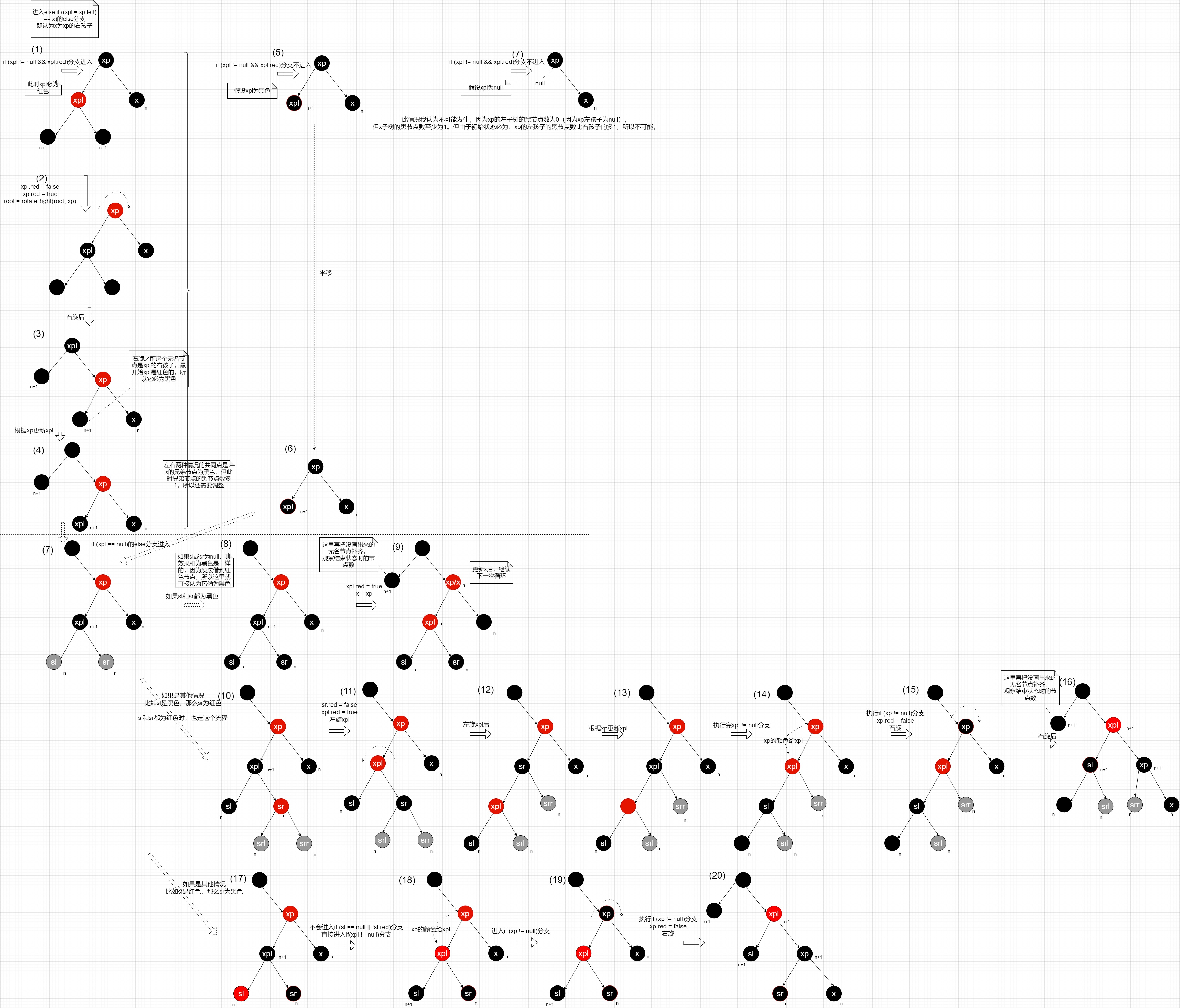• if (xpl != null && xpl.red)这个分支可能执行，可能不执行。

• 如果xpl为红色，那么则会进入此if (xpl != null && xpl.red)分支，如下图所示。如果xpl为红色，那么xp和xpl的孩子的颜色都必为黑色节点。而之前说过，刚开始时x的黑节点数，比x的兄弟节点饿黑节点数少1，我们假设x的黑节点数为n，那么xpl作为它的兄弟节点，xpl的黑节点数则为n+1，由于xpl是红色的不属于黑色节点，那么可推理出xpl的两个孩子的黑节点数也为n+1。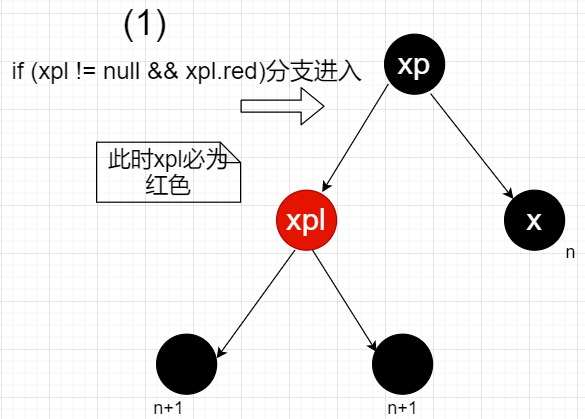• 如果xpl为红色，且执行完if (xpl != null && xpl.red)分支后，如下图所示。调整后，x的兄弟节点变成了一个黑色节点。对比上下图发现，通过旋转操作后，使得x和一个黑节点数为n+1的黑色节点成为了兄弟。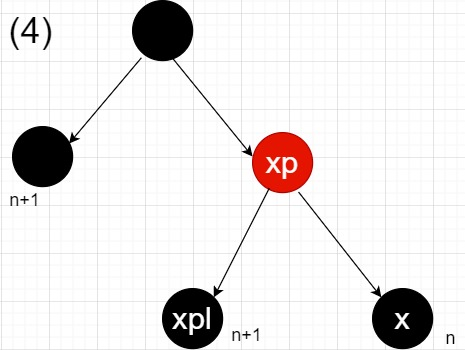• 如果xpl为黑色，那么则不会进入此if (xpl != null && xpl.red)分支，如下图所示。xpl的黑节点数为n+1，比x多1。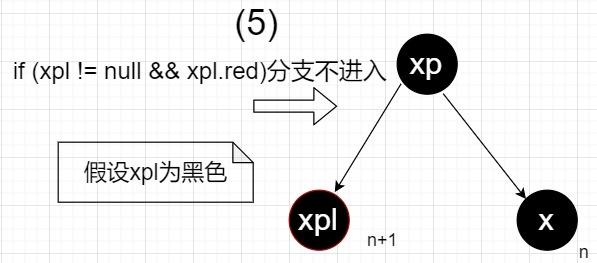• 对比如果xpl为红色，和如果xpl为黑色的两种情况的最终结果，如下图所示，可以发现两种情况最终结果的共同点是：x的兄弟节点必为黑色，但此时兄弟节点的黑节点数多1，所以还需要调整。而两种情况的差异点是：xp的颜色。这也是后面要执行xpl.red = (xp == null) ? false : xp.red（把xp的颜色赋给xpl）的原因。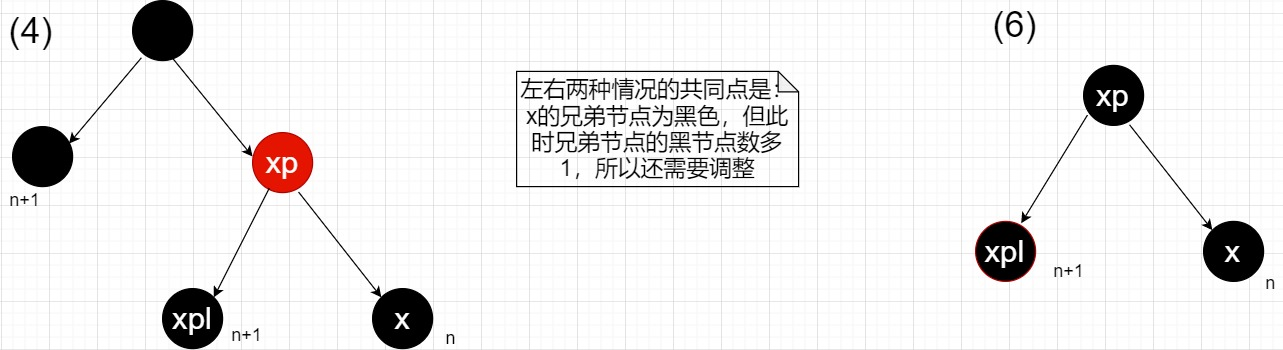• 如果xpl为null，那么则不会进入此if (xpl != null && xpl.red)分支，如下图所示。我认为此分支不可能进入。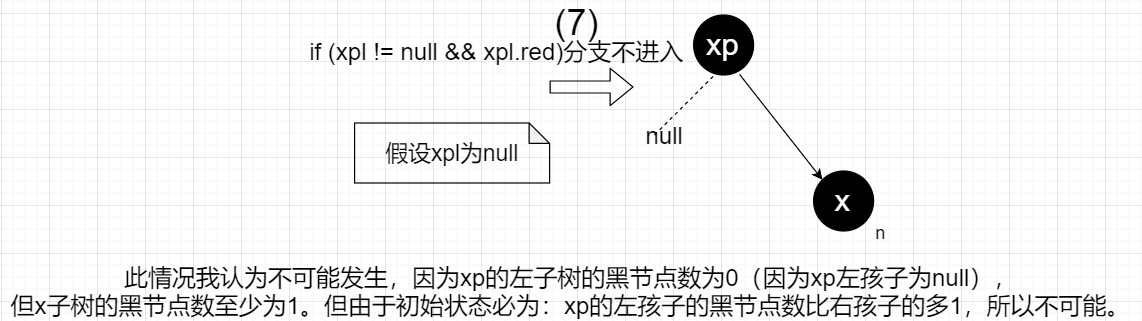• 接下来讲解if (xpl == null)的else分支里的逻辑（根据上一条分析，所以是认为不可能进入if (xpl == null)分支的），在大图中是虚线以下的过程。

• 虚线下的过程，只能操作到x节点，xp节点（x的父节点），xpl节点（x的兄弟节点），sl节点（x的兄弟节点的左孩子）和sr节点（x的兄弟节点的右孩子），即只能操作这上下三层节点。这也是为什么虚线上的过程最后总会调整为xpl节点为黑色节点的情况，因为这样的话，xpl节点的两个孩子sl和sr的黑节点数就为n，而x节点本身的黑节点数也为n。只有找到了黑节点数都为n的节点们后，才方便进行调整，那之后就根据各种情况来再平衡就好了。

• if (xpl == null)的else分支的初始状态如下图（注意，此初始状态是从过程$(4)$而来的，所以虚线下的过程都是过程$(4)$接下来的过程。其实还可以画出从过程$(6)$而来的初始状态，但不必画出了）。由于xpl的黑节点数为n+1，则它自身为黑色，所以推理出，它的左右孩子的黑节点则为n。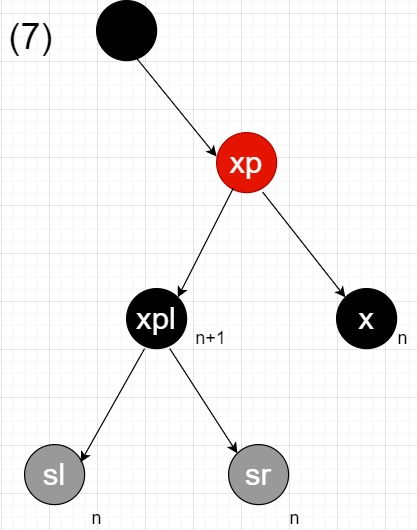• 很有必要说明一下if ((sl == null || !sl.red) && (sr == null || !sr.red))分支和它的else分支的各种情况，如下图所示，它的else分支里，sl和sr中必有一个节点是红色的。而且在else分支里，当sr为红色时，必然还会进入if (sl == null || !sl.red)子分支。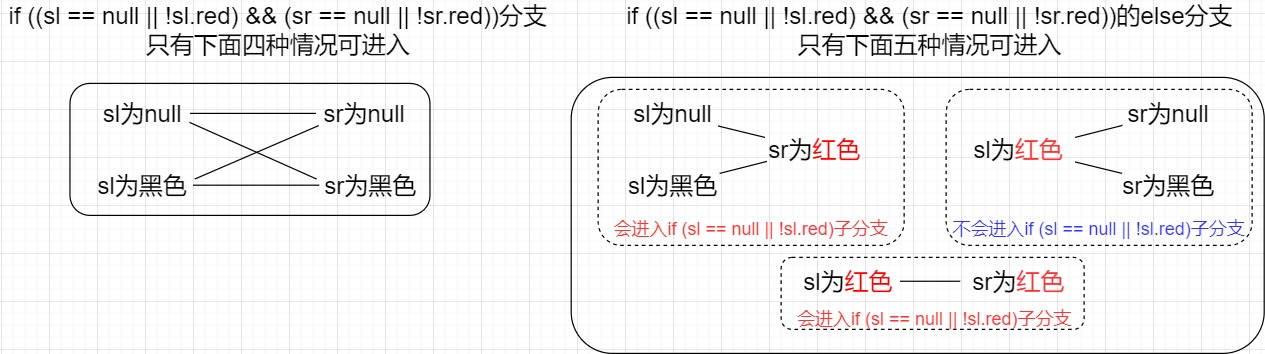• 如果进入了if ((sl == null || !sl.red) && (sr == null || !sr.red))分支，如下图所示。那么说明“sl为null或sl为黑色”和“sr为null或sr为黑色”这两件事都成立，可见过程$(8)$时，x的兄弟节点的两个孩子都是黑色节点，这样的话根本没有操作空间使得x和x的兄弟节点平衡（但凡x的兄弟节点的两个孩子有一个红色节点，也不至于这样）。过程$(9)$里，所以只好另xpl为红色，这样xpl和它的兄弟节点平衡了（黑节点数一样），但由于这里是通过让xpl的黑节点数少1来使得平衡的，且xp的颜色我们又没有变过（这里考虑了虚线上的两种情况的差异点，即xp刚开始的颜色都有可能），所以不管xp的初始颜色是什么，xp必然比xp的兄弟节点的黑节点数少1，所以还是不平衡的，然后继续循环。如果考虑xp初始为黑色，那么过程$(9)$里，xp的黑节点数为n+1，xp的兄弟节点的黑节点数为n+2。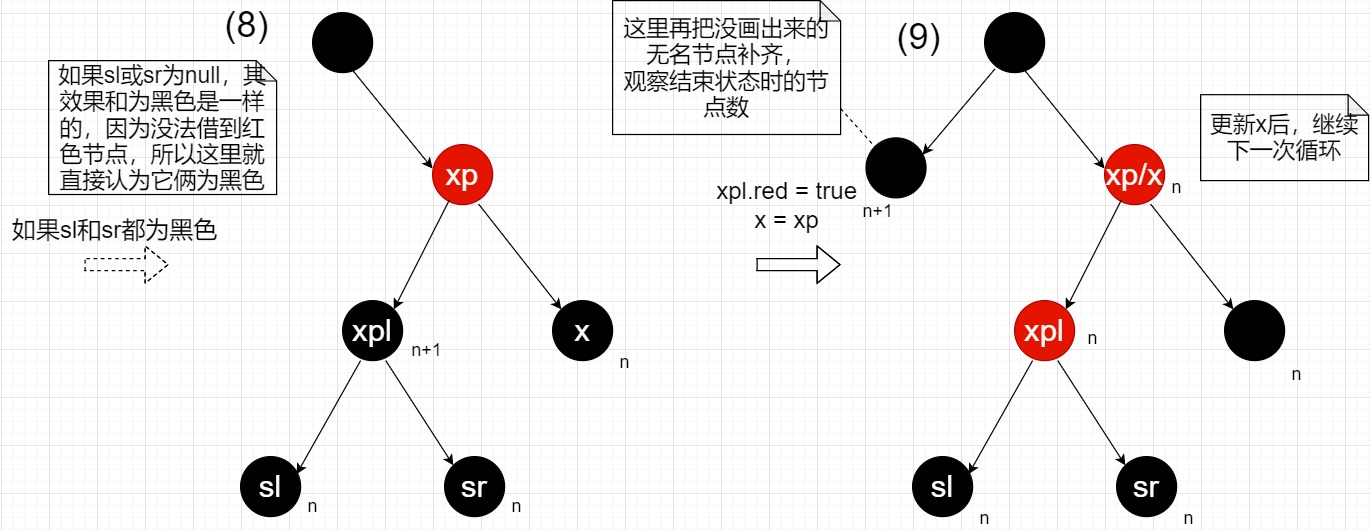• 如果进入了if ((sl == null || !sl.red) && (sr == null || !sr.red))的else分支，如下图所示。那么说明“sl为null或sl为黑色”和“sr为null或sr为黑色”这两件事不是都成立的。观察逻辑可以发现，else分支里可以分为两种情况：1.如果sr为红色，此时不管sl的颜色。 2.如果sr为黑色，sl为红色。其实这两种情况的共同点就是sr和sl中至少有一个红色节点了。

• 假设情况为“如果sr为红色，此时不管sl的颜色”，因为此时sl的颜色无论为什么对过程不会有影响。如下图所示，为这种情况的开始过程和结束过程。发现过程$(16)$时，整个树已经平衡了，结束后会将x指向root（x = root），下次循环就会直接退出啦。且过程$(10)$里xp这个位置，对应到过程$(16)$里则变成了xpl这个节点，且过程$(10)$里xp的颜色还可能为黑色，那么过程$(16)$的xpl会和过程$(10)$里xp的颜色一致（虚线下的三行过程都保证了这一点）。这是通过将xp的颜色赋给xpl（xpl.red = (xp == null) ? false : xp.red），再右旋xp（rotateRight(root, xp)）来保证的，这样，就把虚线上的差异点考虑在内了。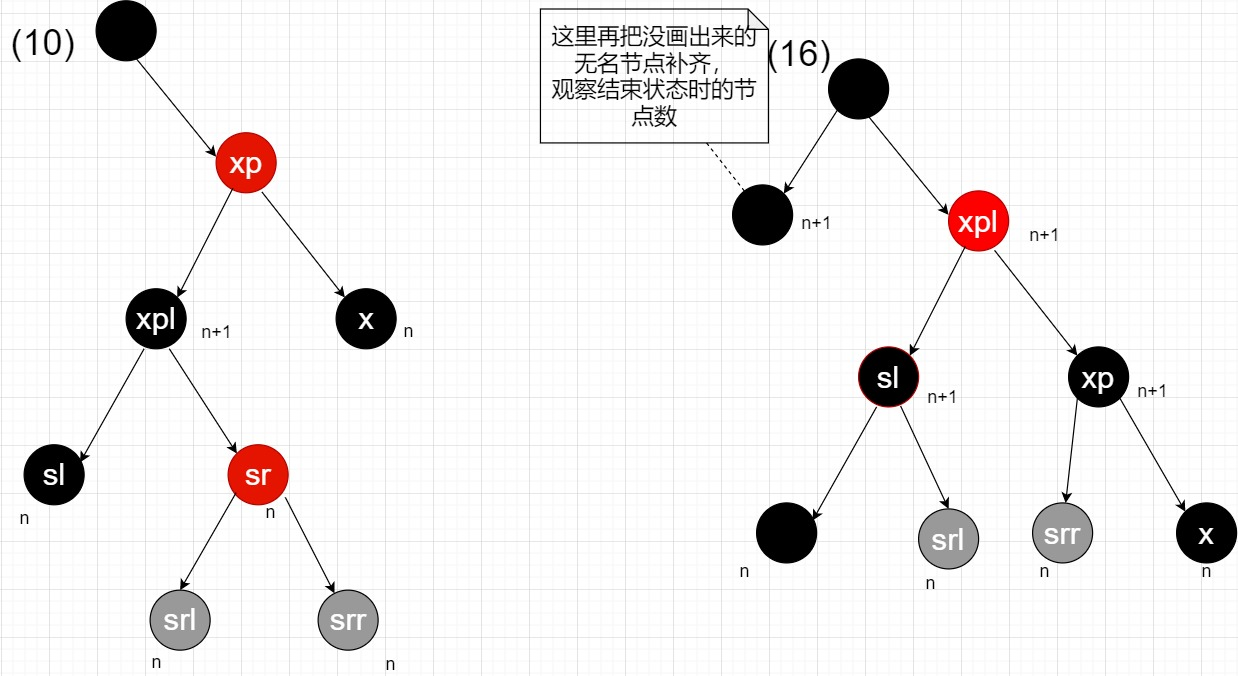• 再假设情况为“如果sr为黑色，sl为红色”，如下图所示，为这种情况的开始过程和结束过程。发现过程$(20)$时，整个树已经平衡了，结束后会将x指向root（x = root），下次循环就会直接退出啦。同样的，过程$(17)$里xp这个位置对应过过程$(20)$里会保持相同位置的节点颜色一致。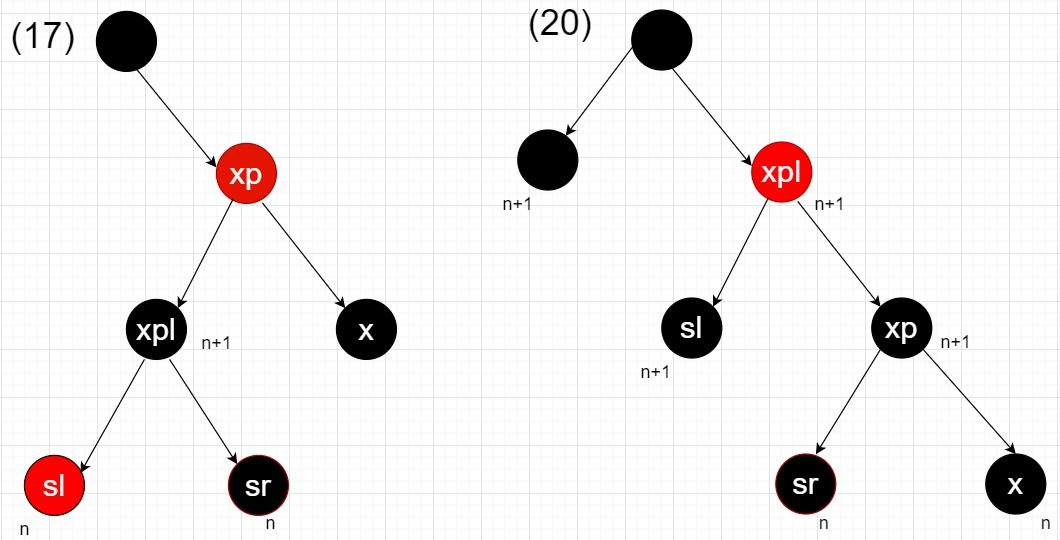• 虚线下的第二行过程（过程$(10)$到过程$(16)$）和第三行过程（过程$(17)$到过程$(20)$），除了开始过程和结束过程外，中间过程里我只给那些调整过程中黑节点数不变的节点标注出来了黑节点数，其他没有标注出来的节点只需要在结束过程里进行确认就好了。

• 之所以虚线下的第二行过程和第三行过程要进行区分，是因为sr是否为红色，需要进行的调整操作是不一样的。比如过程过程$(10)$如果走的是第三行过程的流程，如下图所示，最终会造成sl和xp这两个兄弟节点不是平衡的。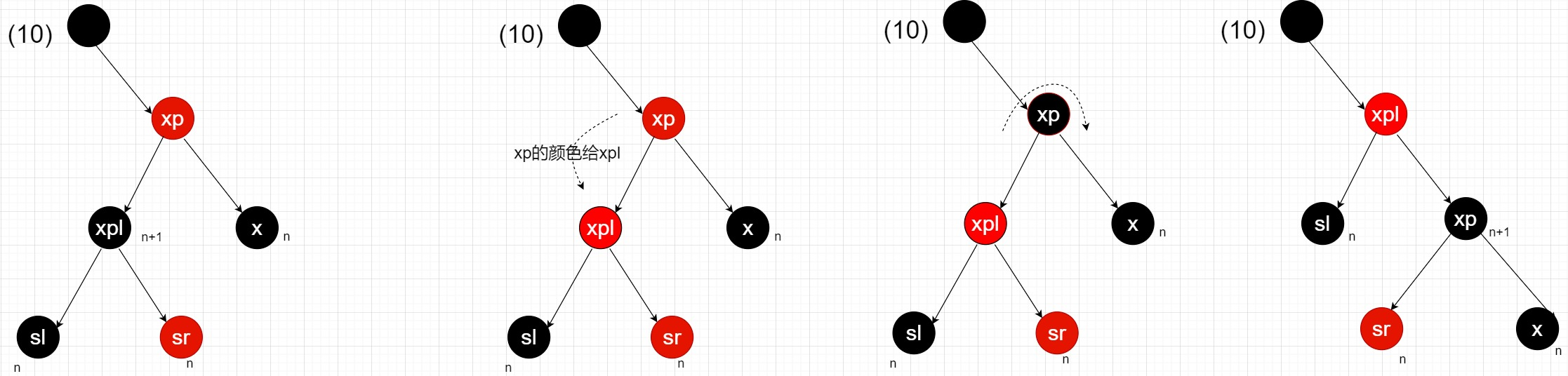• 和balanceInsertion一样，此balanceDeletion函数同样只处理三层树的结构。
• 每次循环体里，除非进入那些直接return的终点，那么循环体开始时，x节点总是比x节点的兄弟节点的黑节点数少1的。
• 虚线下的过程，其主要技巧(指的是虚线下第二行和第三行。第一行是先让自己和兄弟平衡，但却是通过不是让自己加1，而是让兄弟减1，所以还需要x往上移动，往更高层借红色节点)是通过借用颜色为红色的兄弟节点的左右孩子，只要有一个孩子是红色的，就可以借用。而借用其实就是，通过旋转操作把红色节点弄到自己的子树里，然后通过红色变黑色，让自己子树的黑节点数加1，从达到平衡。
• 大图中，到达虚线时的过程，x的兄弟节点总会是黑色的。根据前提“x节点总是比x节点的兄弟节点的黑节点数少1”，而兄弟节点又是黑色，可以推理出“x的兄弟节点的两个孩子的黑节点数，和x节点一样大”，找到了一样大的节点，之后才好处理。

# checkInvariants

        static <K,V> boolean checkInvariants(TreeNode<K,V> t) {
TreeNode<K,V> tp = t.parent, tl = t.left, tr = t.right,
tb = t.prev, tn = (TreeNode<K,V>)t.next;
//检查双向链表的结构是否正确
if (tb != null && tb.next != t)
return false;
if (tn != null && tn.prev != t)
return false;
//检查红黑树的结构是否正确
if (tp != null && t != tp.left && t != tp.right)
return false;
if (tl != null && (tl.parent != t || tl.hash > t.hash))
return false;
if (tr != null && (tr.parent != t || tr.hash < t.hash))
return false;
//检查红黑树的颜色是否正确
if (t.red && tl != null && tl.red && tr != null && tr.red)
return false;
//递归调用自身，检查左右孩子
if (tl != null && !checkInvariants(tl))
return false;
if (tr != null && !checkInvariants(tr))
return false;
return true;
}

• 这是一个递归函数。整个执行过程类似于二叉树，叶子节点返回的bool值给上一层，以此类推，一直到了root。
• 返回上一层后，if (tl != null && !checkInvariants(tl))if (tr != null && !checkInvariants(tr))会分别判断两个底层返回来的bool值，如果返回的bool值都是true，那么将执行终点return true

©️2019 CSDN 皮肤主题: 精致技术 设计师: CSDN官方博客